Скачать презентацию Optimization Compilers Modern compilers operate under several

4176e1e942a74a3e2734fa09384906aa.ppt

• Количество слайдов: 72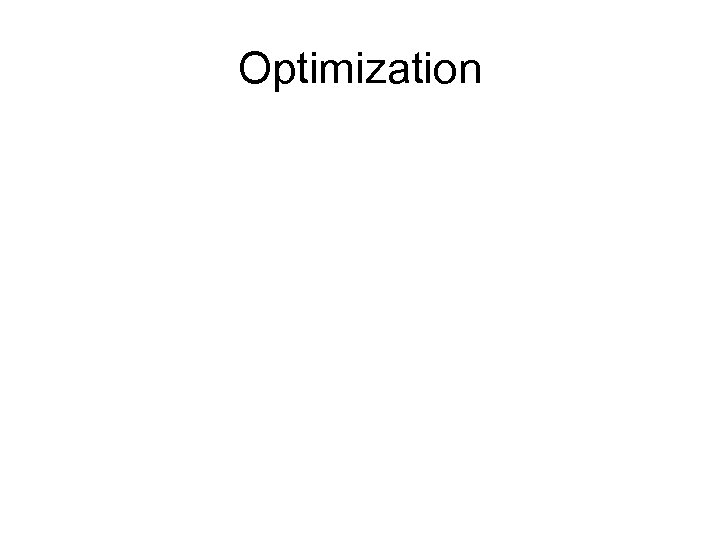Optimization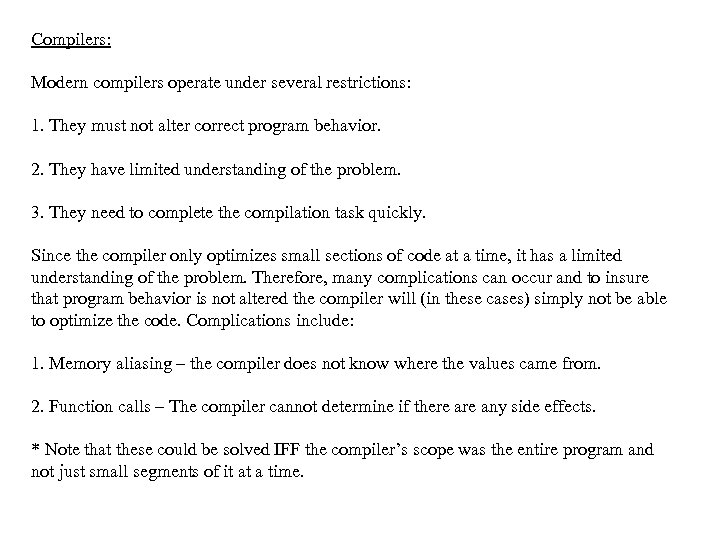Compilers: Modern compilers operate under several restrictions: 1. They must not alter correct program behavior. 2. They have limited understanding of the problem. 3. They need to complete the compilation task quickly. Since the compiler only optimizes small sections of code at a time, it has a limited understanding of the problem. Therefore, many complications can occur and to insure that program behavior is not altered the compiler will (in these cases) simply not be able to optimize the code. Complications include: 1. Memory aliasing – the compiler does not know where the values came from. 2. Function calls – The compiler cannot determine if there any side effects. * Note that these could be solved IFF the compiler’s scope was the entire program and not just small segments of it at a time.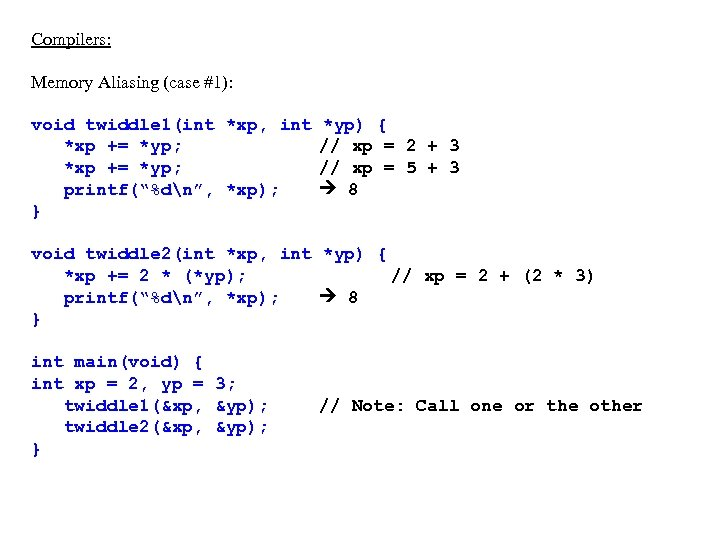Compilers: Memory Aliasing (case #1): void twiddle 1(int *xp, int *xp += *yp; printf(“%dn”, *xp); } *yp) { // xp = 2 + 3 // xp = 5 + 3 8 void twiddle 2(int *xp, int *yp) { *xp += 2 * (*yp); // xp = 2 + (2 * 3) printf(“%dn”, *xp); 8 } int main(void) { int xp = 2, yp = 3; twiddle 1(&xp, &yp); twiddle 2(&xp, &yp); } // Note: Call one or the other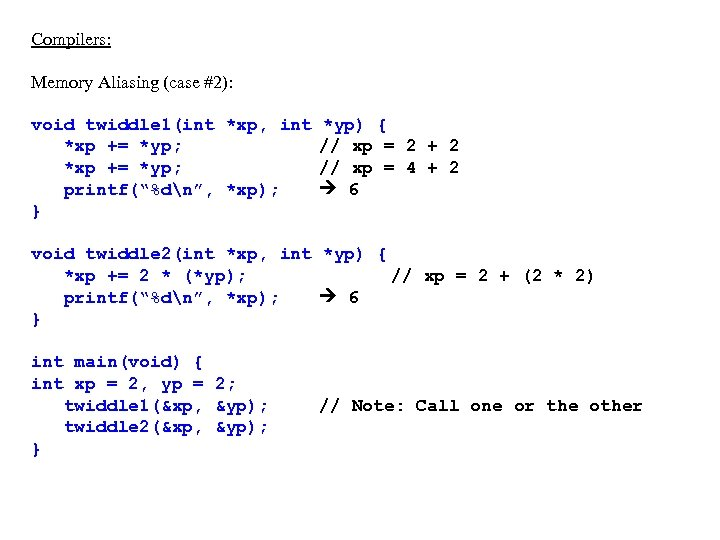Compilers: Memory Aliasing (case #2): void twiddle 1(int *xp, int *xp += *yp; printf(“%dn”, *xp); } *yp) { // xp = 2 + 2 // xp = 4 + 2 6 void twiddle 2(int *xp, int *yp) { *xp += 2 * (*yp); // xp = 2 + (2 * 2) printf(“%dn”, *xp); 6 } int main(void) { int xp = 2, yp = 2; twiddle 1(&xp, &yp); twiddle 2(&xp, &yp); } // Note: Call one or the other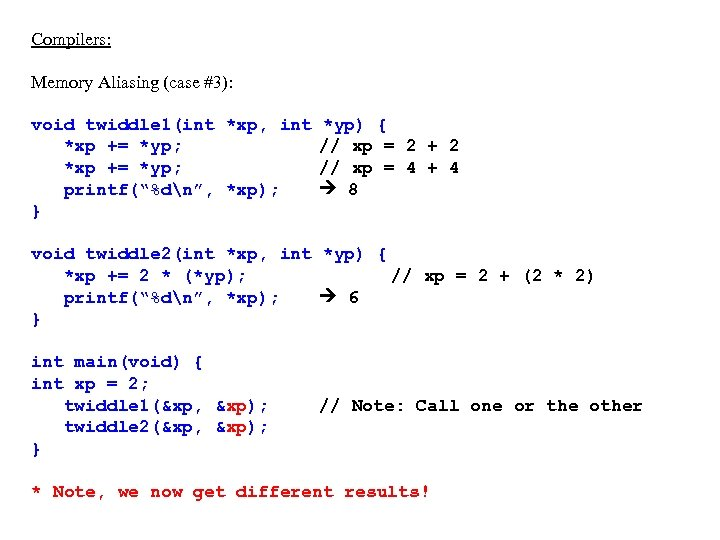Compilers: Memory Aliasing (case #3): void twiddle 1(int *xp, int *xp += *yp; printf(“%dn”, *xp); } *yp) { // xp = 2 + 2 // xp = 4 + 4 8 void twiddle 2(int *xp, int *yp) { *xp += 2 * (*yp); // xp = 2 + (2 * 2) printf(“%dn”, *xp); 6 } int main(void) { int xp = 2; twiddle 1(&xp, &xp); twiddle 2(&xp, &xp); } // Note: Call one or the other * Note, we now get different results!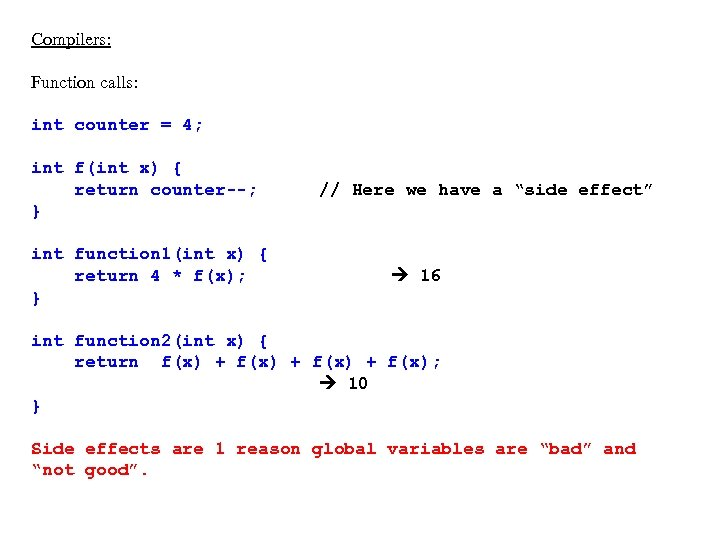Compilers: Function calls: int counter = 4; int f(int x) { return counter--; } int function 1(int x) { return 4 * f(x); } // Here we have a “side effect” 16 int function 2(int x) { return f(x) + f(x); 10 } Side effects are 1 reason global variables are “bad” and “not good”.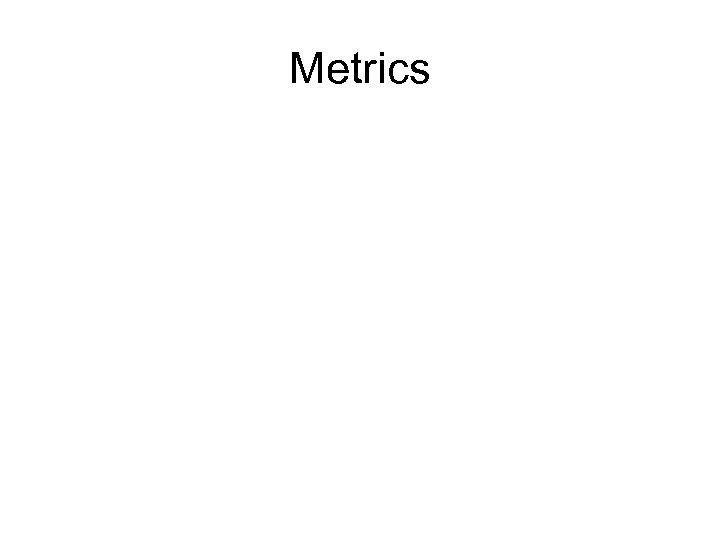Metrics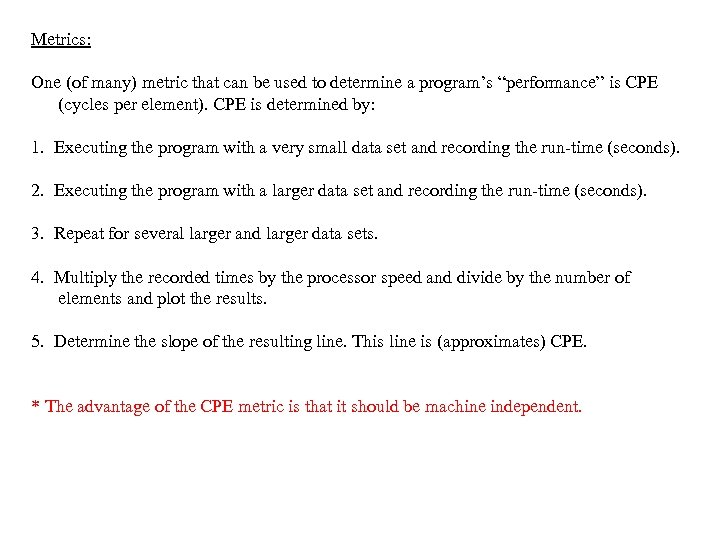Metrics: One (of many) metric that can be used to determine a program’s “performance” is CPE (cycles per element). CPE is determined by: 1. Executing the program with a very small data set and recording the run-time (seconds). 2. Executing the program with a larger data set and recording the run-time (seconds). 3. Repeat for several larger and larger data sets. 4. Multiply the recorded times by the processor speed and divide by the number of elements and plot the results. 5. Determine the slope of the resulting line. This line is (approximates) CPE. * The advantage of the CPE metric is that it should be machine independent.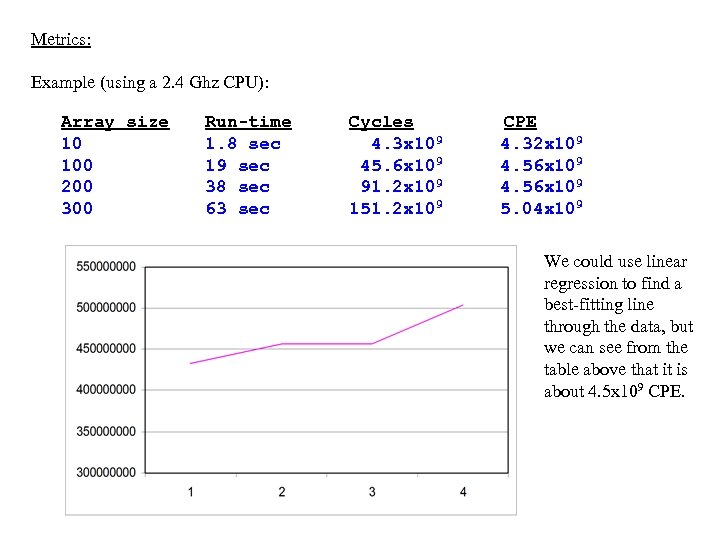Metrics: Example (using a 2. 4 Ghz CPU): Array size 10 100 200 300 Run-time 1. 8 sec 19 sec 38 sec 63 sec Cycles 4. 3 x 109 45. 6 x 109 91. 2 x 109 151. 2 x 109 CPE 4. 32 x 109 4. 56 x 109 5. 04 x 109 We could use linear regression to find a best-fitting line through the data, but we can see from the table above that it is about 4. 5 x 109 CPE.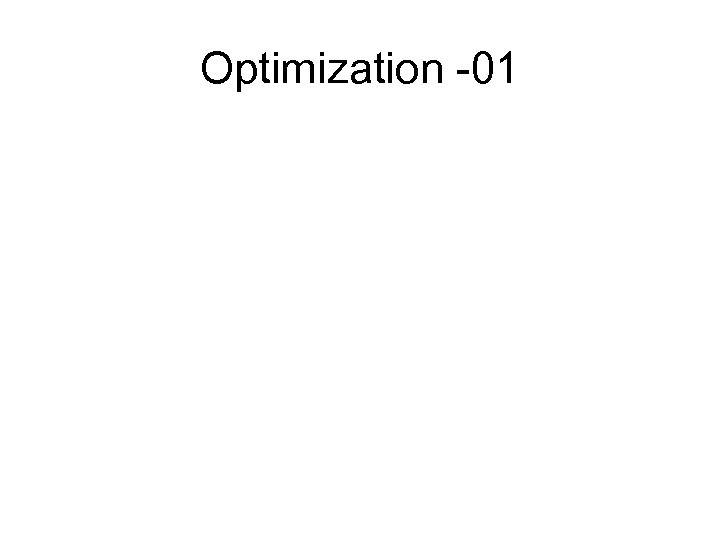Optimization -01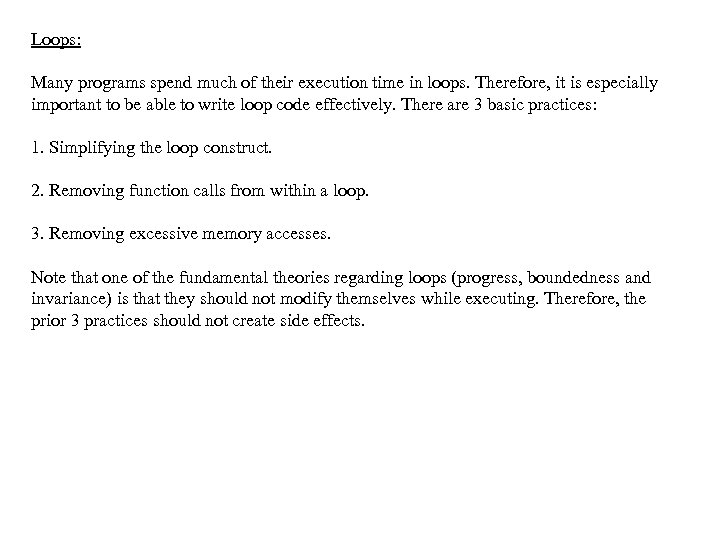Loops: Many programs spend much of their execution time in loops. Therefore, it is especially important to be able to write loop code effectively. There are 3 basic practices: 1. Simplifying the loop construct. 2. Removing function calls from within a loop. 3. Removing excessive memory accesses. Note that one of the fundamental theories regarding loops (progress, boundedness and invariance) is that they should not modify themselves while executing. Therefore, the prior 3 practices should not create side effects.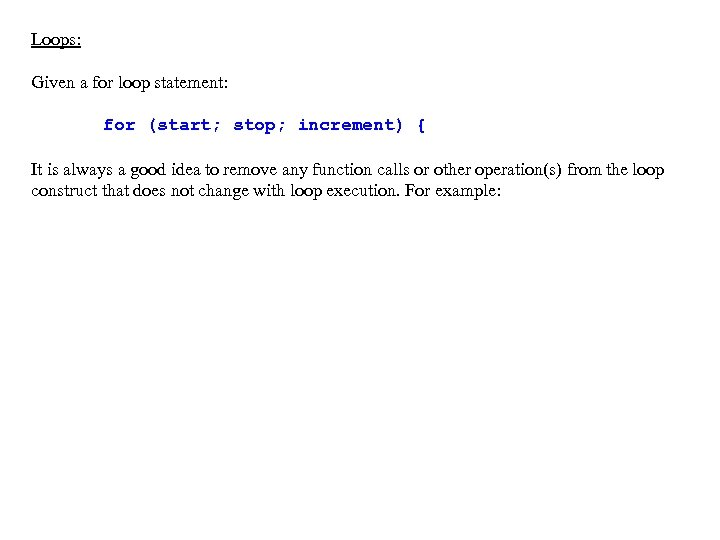Loops: Given a for loop statement: for (start; stop; increment) { It is always a good idea to remove any function calls or other operation(s) from the loop construct that does not change with loop execution. For example: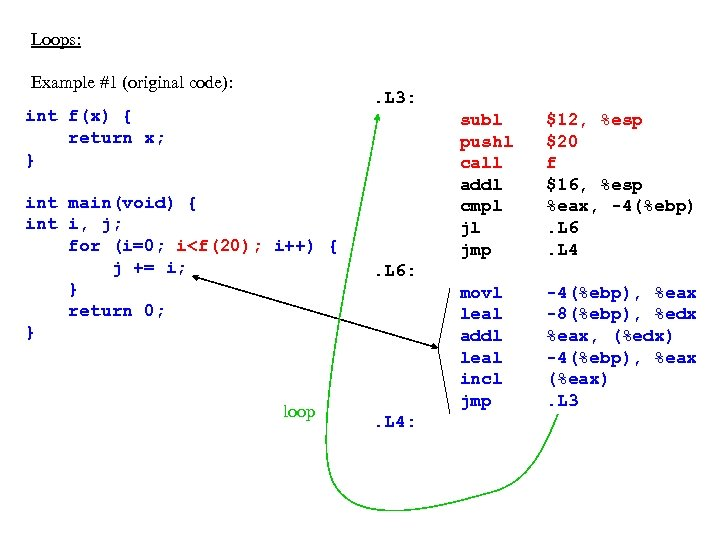Loops: Example #1 (original code): . L 3: int f(x) { return x; } int main(void) { int i, j; for (i=0; i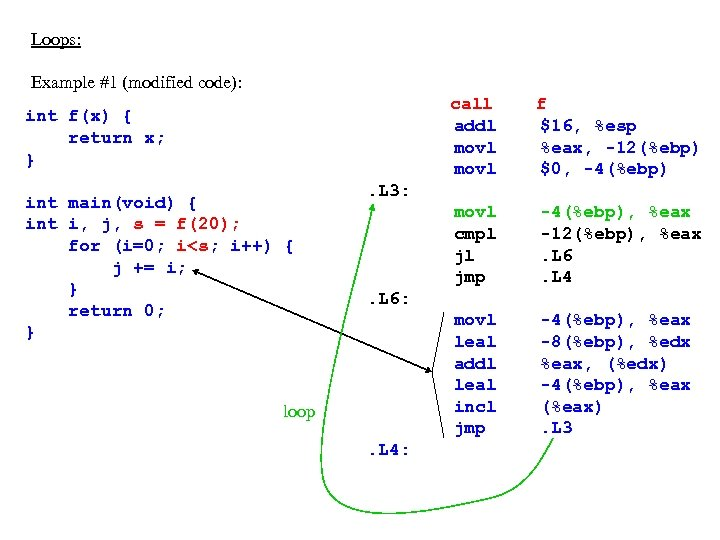Loops: Example #1 (modified code): call addl movl int main(void) { int i, j, s = f(20); for (i=0; i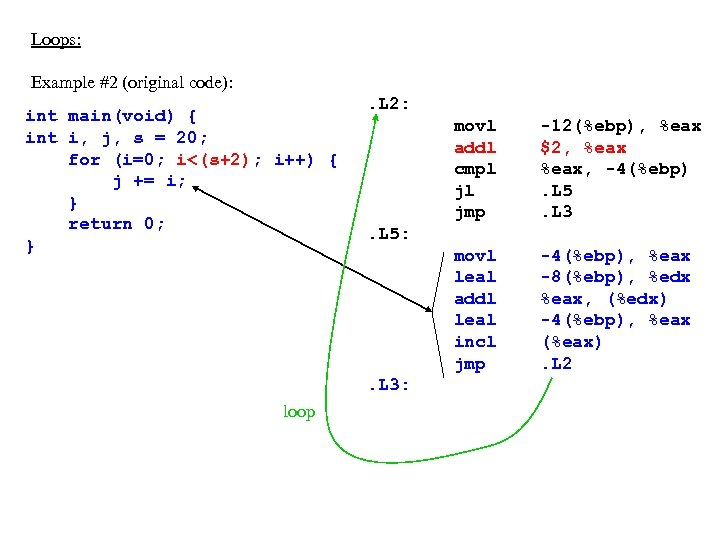Loops: Example #2 (original code): int main(void) { int i, j, s = 20; for (i=0; i<(s+2); i++) { j += i; } return 0; } . L 2: movl addl cmpl jl jmp movl leal addl leal incl jmp -4(%ebp), %eax -8(%ebp), %edx %eax, (%edx) -4(%ebp), %eax (%eax). L 2 . L 5: . L 3: loop -12(%ebp), %eax \$2, %eax, -4(%ebp). L 5. L 3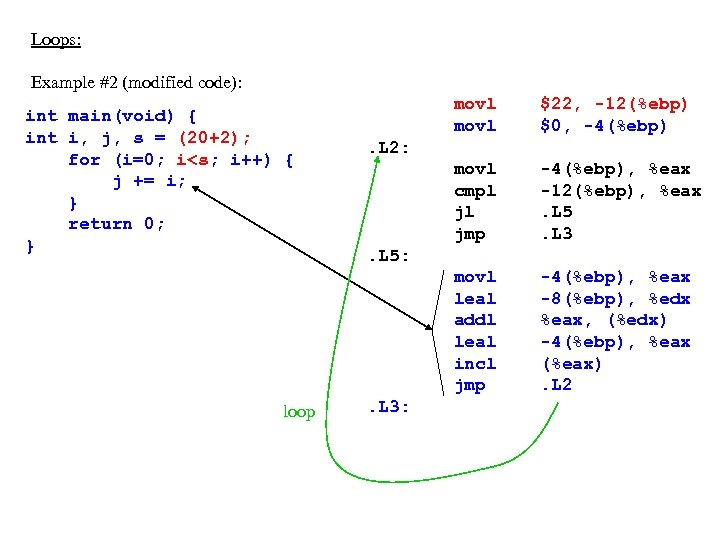Loops: Example #2 (modified code): loop movl \$22, -12(%ebp) \$0, -4(%ebp) movl cmpl jl jmp -4(%ebp), %eax -12(%ebp), %eax. L 5. L 3 movl leal addl leal incl jmp int main(void) { int i, j, s = (20+2); for (i=0; i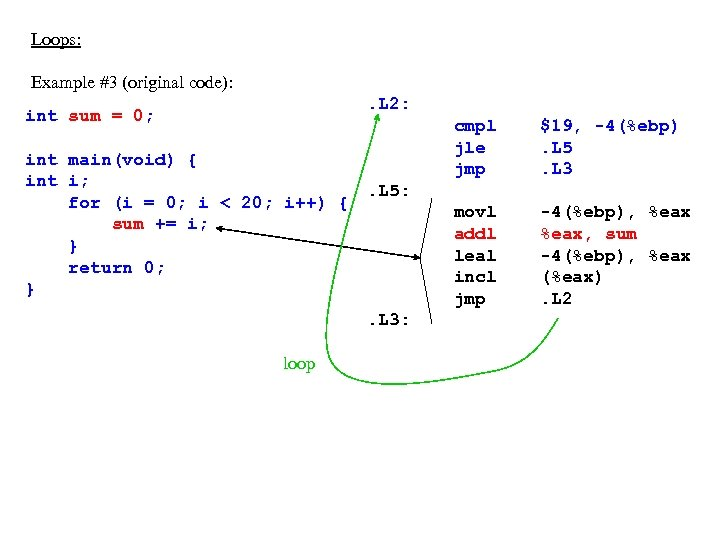Loops: Example #3 (original code): . L 2: int sum = 0; int main(void) { int i; for (i = 0; i < 20; i++) { sum += i; } return 0; } cmpl jle jmp movl addl leal incl jmp -4(%ebp), %eax, sum -4(%ebp), %eax (%eax). L 2 . L 5: . L 3: loop \$19, -4(%ebp). L 5. L 3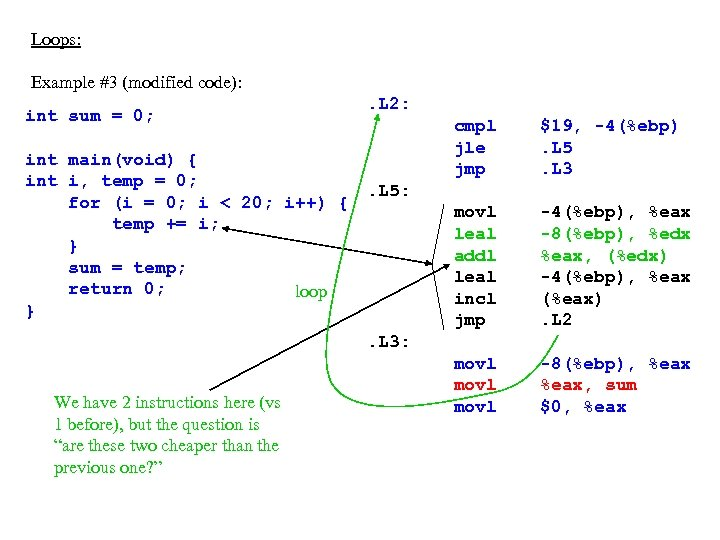Loops: Example #3 (modified code): int sum = 0; int main(void) { int i, temp = 0; for (i = 0; i < 20; i++) { temp += i; } sum = temp; return 0; loop } . L 2: cmpl jle jmp \$19, -4(%ebp). L 5. L 3 movl leal addl leal incl jmp -4(%ebp), %eax -8(%ebp), %edx %eax, (%edx) -4(%ebp), %eax (%eax). L 2 movl -8(%ebp), %eax, sum \$0, %eax . L 5: . L 3: We have 2 instructions here (vs 1 before), but the question is “are these two cheaper than the previous one? ”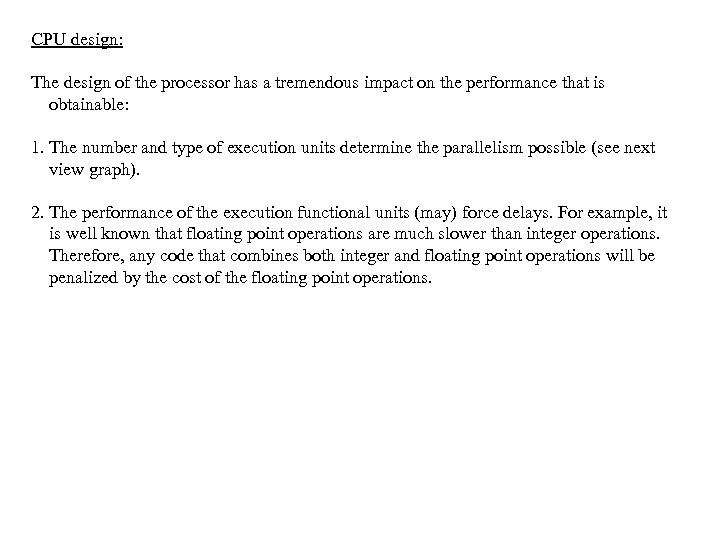CPU design: The design of the processor has a tremendous impact on the performance that is obtainable: 1. The number and type of execution units determine the parallelism possible (see next view graph). 2. The performance of the execution functional units (may) force delays. For example, it is well known that floating point operations are much slower than integer operations. Therefore, any code that combines both integer and floating point operations will be penalized by the cost of the floating point operations.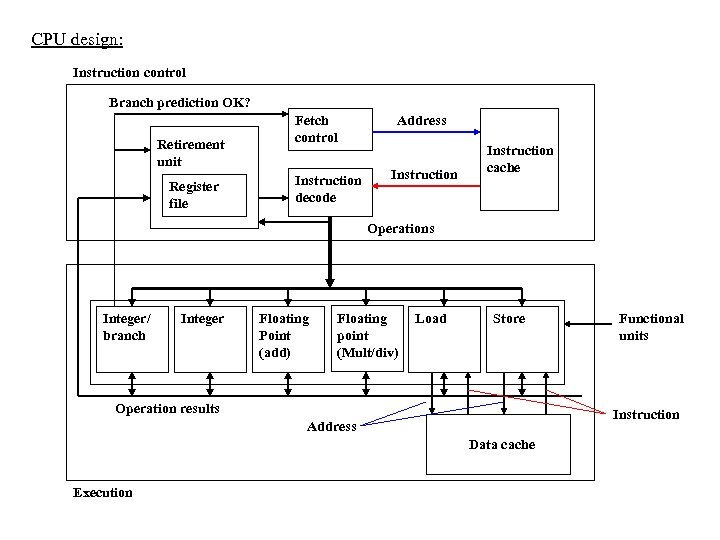CPU design: Instruction control Branch prediction OK? Retirement unit Register file Fetch control Instruction decode Address Instruction cache Operations Integer/ branch Integer Floating Point (add) Floating point (Mult/div) Load Store Operation results Instruction Address Data cache Execution Functional units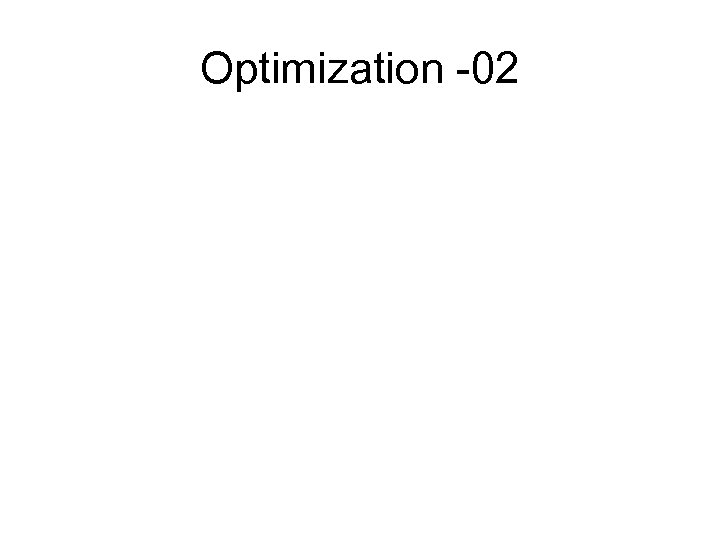Optimization -02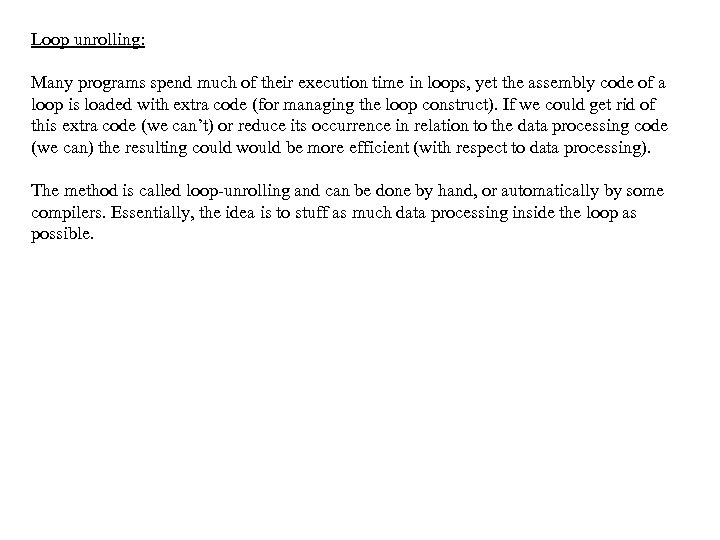Loop unrolling: Many programs spend much of their execution time in loops, yet the assembly code of a loop is loaded with extra code (for managing the loop construct). If we could get rid of this extra code (we can’t) or reduce its occurrence in relation to the data processing code (we can) the resulting could would be more efficient (with respect to data processing). The method is called loop-unrolling and can be done by hand, or automatically by some compilers. Essentially, the idea is to stuff as much data processing inside the loop as possible.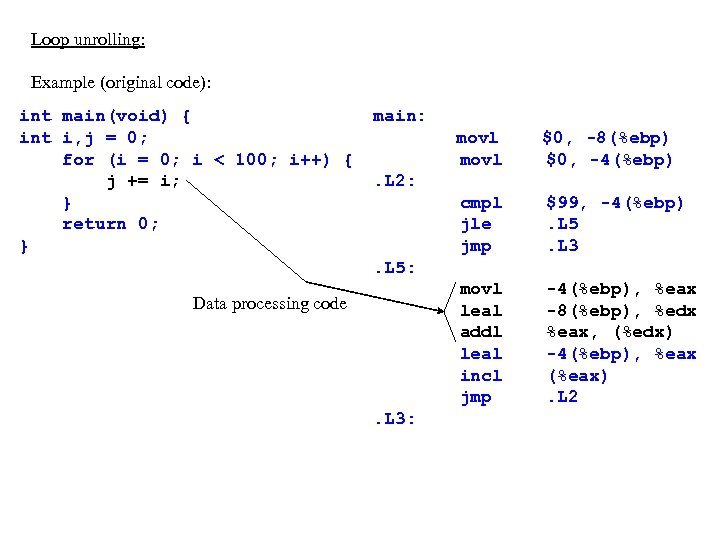Loop unrolling: Example (original code): int main(void) { int i, j = 0; for (i = 0; i < 100; i++) { j += i; } return 0; } main: movl \$0, -8(%ebp) \$0, -4(%ebp) cmpl jle jmp \$99, -4(%ebp). L 5. L 3 movl leal addl leal incl jmp -4(%ebp), %eax -8(%ebp), %edx %eax, (%edx) -4(%ebp), %eax (%eax). L 2: . L 5: Data processing code . L 3: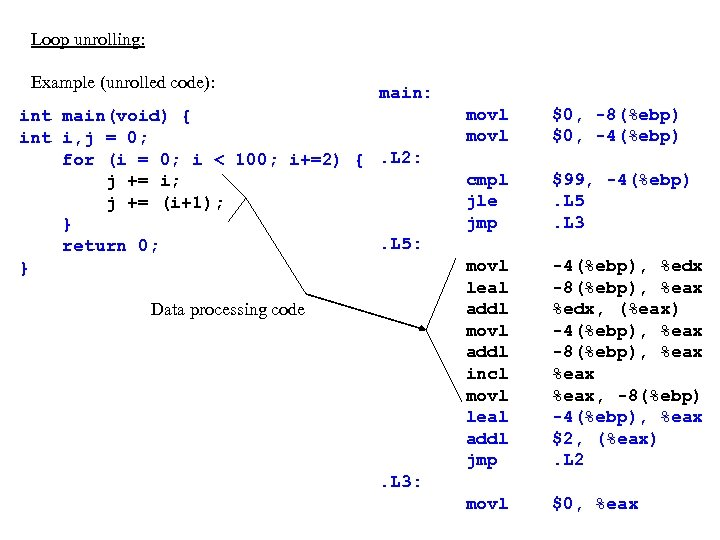Loop unrolling: Example (unrolled code): main: int main(void) { int i, j = 0; for (i = 0; i < 100; i+=2) {. L 2: j += i; j += (i+1); }. L 5: return 0; } Data processing code movl \$0, -8(%ebp) \$0, -4(%ebp) cmpl jle jmp \$99, -4(%ebp). L 5. L 3 movl leal addl movl addl incl movl leal addl jmp -4(%ebp), %edx -8(%ebp), %eax %edx, (%eax) -4(%ebp), %eax -8(%ebp), %eax, -8(%ebp) -4(%ebp), %eax \$2, (%eax). L 2 movl \$0, %eax . L 3: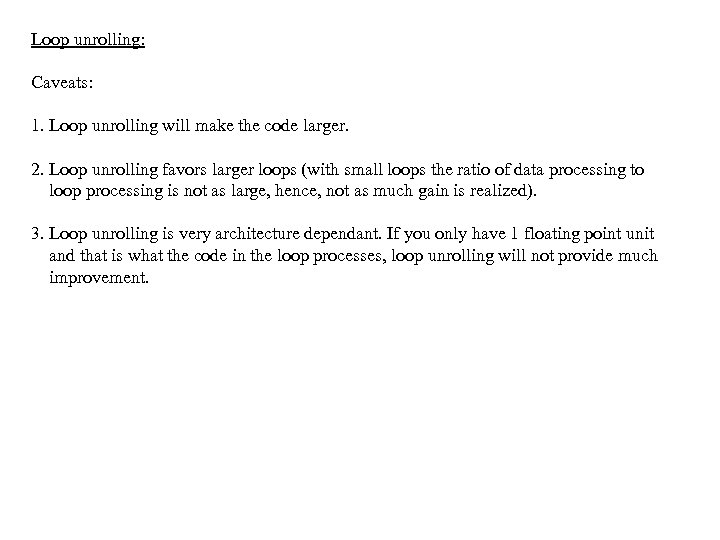Loop unrolling: Caveats: 1. Loop unrolling will make the code larger. 2. Loop unrolling favors larger loops (with small loops the ratio of data processing to loop processing is not as large, hence, not as much gain is realized). 3. Loop unrolling is very architecture dependant. If you only have 1 floating point unit and that is what the code in the loop processes, loop unrolling will not provide much improvement.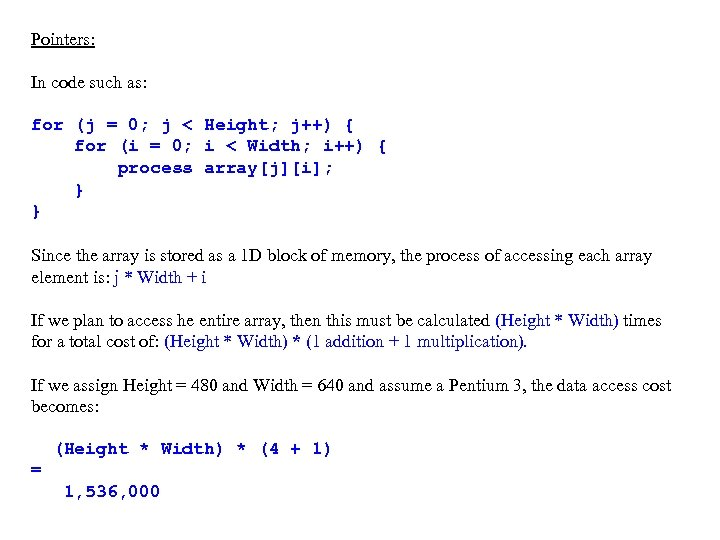Pointers: In code such as: for (j = 0; j < Height; j++) { for (i = 0; i < Width; i++) { process array[j][i]; } } Since the array is stored as a 1 D block of memory, the process of accessing each array element is: j * Width + i If we plan to access he entire array, then this must be calculated (Height * Width) times for a total cost of: (Height * Width) * (1 addition + 1 multiplication). If we assign Height = 480 and Width = 640 and assume a Pentium 3, the data access cost becomes: (Height * Width) * (4 + 1) = 1, 536, 000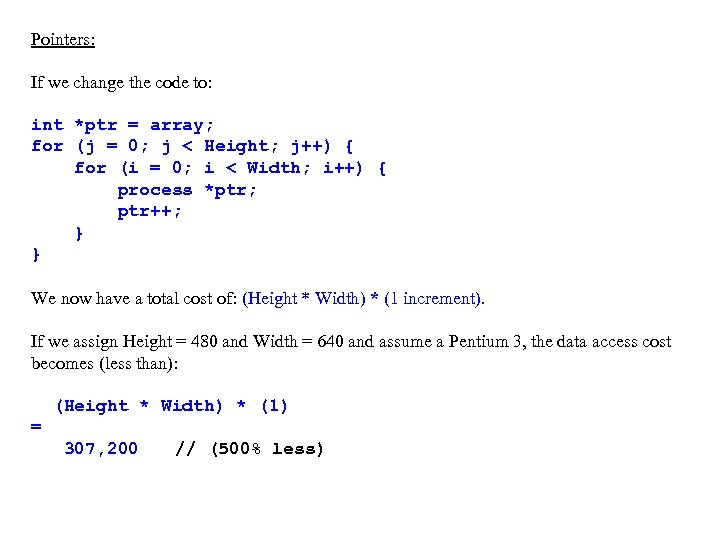Pointers: If we change the code to: int *ptr = array; for (j = 0; j < Height; j++) { for (i = 0; i < Width; i++) { process *ptr; ptr++; } } We now have a total cost of: (Height * Width) * (1 increment). If we assign Height = 480 and Width = 640 and assume a Pentium 3, the data access cost becomes (less than): (Height * Width) * (1) = 307, 200 // (500% less)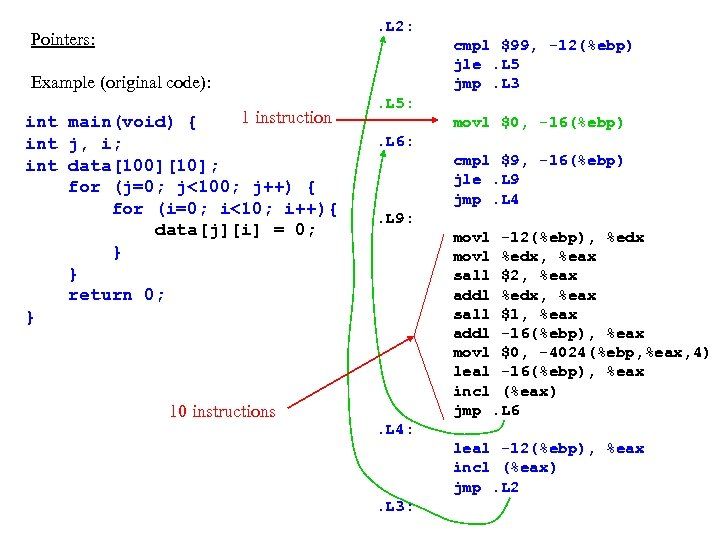. L 2: Pointers: cmpl \$99, -12(%ebp) jle. L 5 jmp. L 3 Example (original code): 1 instruction int main(void) { int j, i; int data; for (j=0; j<100; j++) { for (i=0; i<10; i++){ data[j][i] = 0; } } return 0; } 10 instructions . L 5: movl \$0, -16(%ebp). L 6: cmpl \$9, -16(%ebp) jle. L 9 jmp. L 4. L 9: movl -12(%ebp), %edx movl %edx, %eax sall \$2, %eax addl %edx, %eax sall \$1, %eax addl -16(%ebp), %eax movl \$0, -4024(%ebp, %eax, 4) leal -16(%ebp), %eax incl (%eax) jmp. L 6. L 4: leal -12(%ebp), %eax incl (%eax) jmp. L 2. L 3: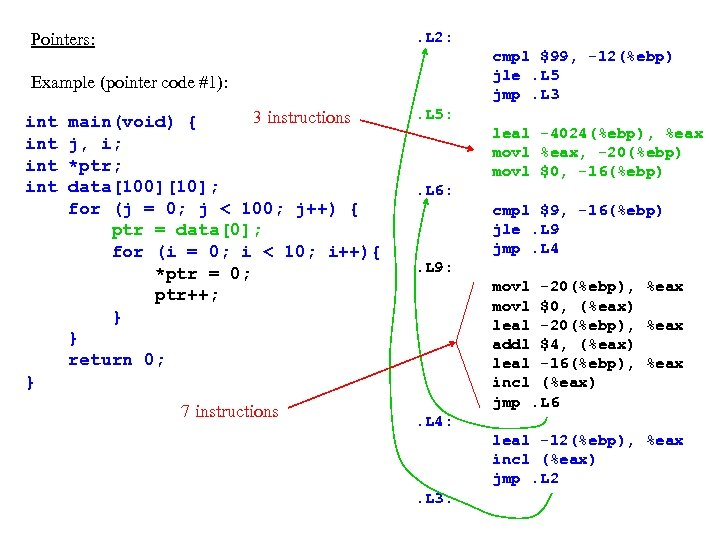. L 2: Pointers: cmpl \$99, -12(%ebp) jle. L 5 jmp. L 3 Example (pointer code #1): int int 3 instructions main(void) { j, i; *ptr; data; for (j = 0; j < 100; j++) { ptr = data; for (i = 0; i < 10; i++){ *ptr = 0; ptr++; } } return 0; . L 5: leal -4024(%ebp), %eax movl %eax, -20(%ebp) movl \$0, -16(%ebp). L 6: cmpl \$9, -16(%ebp) jle. L 9 jmp. L 4. L 9: movl -20(%ebp), %eax movl \$0, (%eax) leal -20(%ebp), %eax addl \$4, (%eax) leal -16(%ebp), %eax incl (%eax) jmp. L 6 } 7 instructions . L 4: leal -12(%ebp), %eax incl (%eax) jmp. L 2. L 3: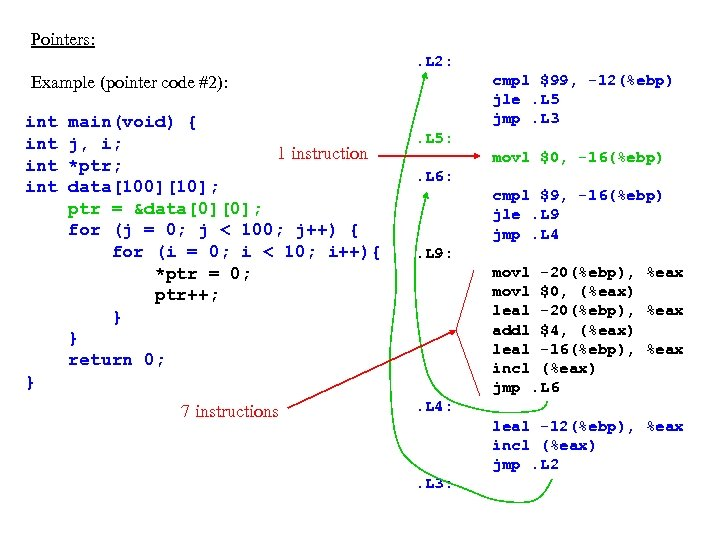Pointers: . L 2: cmpl \$99, -12(%ebp) jle. L 5 jmp. L 3 Example (pointer code #2): int int main(void) { j, i; 1 instruction *ptr; data; ptr = &data; for (j = 0; j < 100; j++) { for (i = 0; i < 10; i++){ *ptr = 0; ptr++; } } return 0; . L 5: movl \$0, -16(%ebp). L 6: cmpl \$9, -16(%ebp) jle. L 9 jmp. L 4. L 9: movl -20(%ebp), %eax movl \$0, (%eax) leal -20(%ebp), %eax addl \$4, (%eax) leal -16(%ebp), %eax incl (%eax) jmp. L 6 } 7 instructions . L 4: leal -12(%ebp), %eax incl (%eax) jmp. L 2. L 3:Pointers: Caveats: 1. Use of pointers makes the code difficult to read. 2. Use of pointers limits the data access method to being sequential.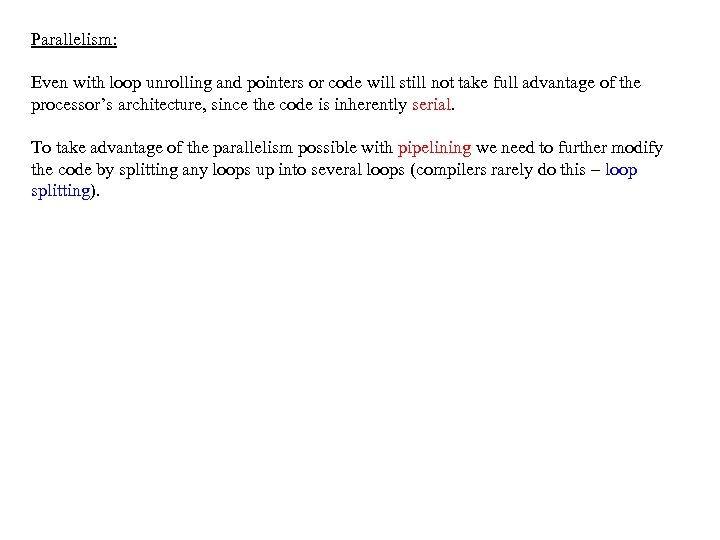Parallelism: Even with loop unrolling and pointers or code will still not take full advantage of the processor’s architecture, since the code is inherently serial. To take advantage of the parallelism possible with pipelining we need to further modify the code by splitting any loops up into several loops (compilers rarely do this – loop splitting).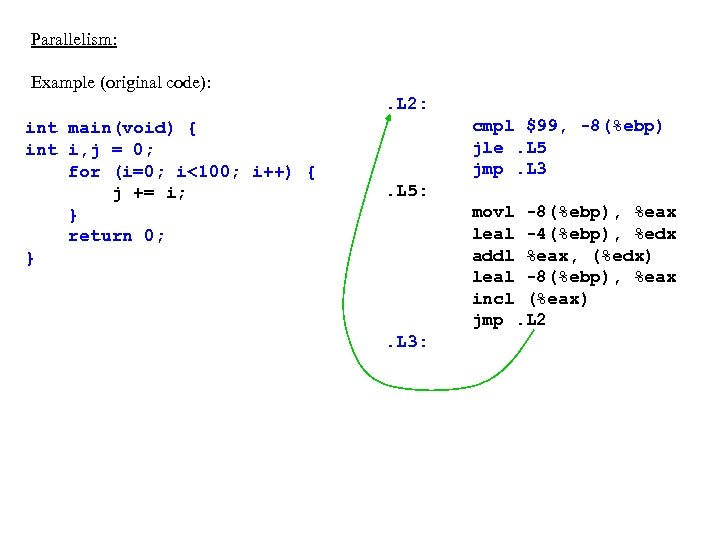Parallelism: Example (original code): . L 2: int main(void) { int i, j = 0; for (i=0; i<100; i++) { j += i; } return 0; } cmpl \$99, -8(%ebp) jle. L 5 jmp. L 3. L 5: movl -8(%ebp), %eax leal -4(%ebp), %edx addl %eax, (%edx) leal -8(%ebp), %eax incl (%eax) jmp. L 2. L 3: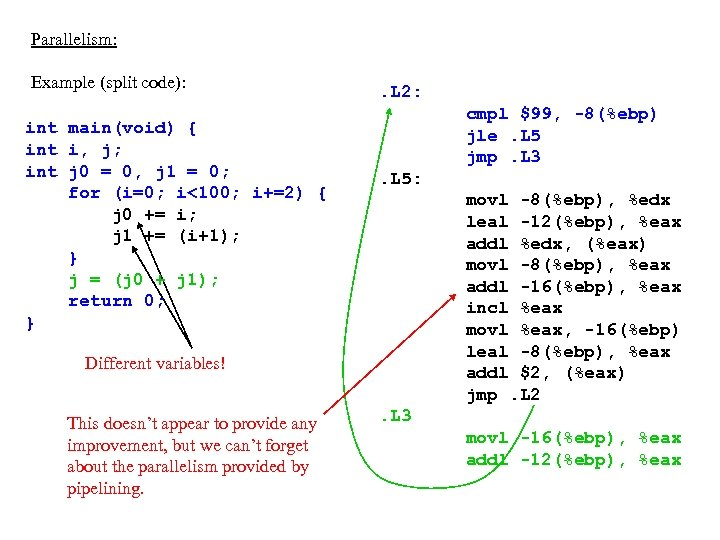Parallelism: Example (split code): int main(void) { int i, j; int j 0 = 0, j 1 = 0; for (i=0; i<100; i+=2) { j 0 += i; j 1 += (i+1); } j = (j 0 + j 1); return 0; } . L 2: cmpl \$99, -8(%ebp) jle. L 5 jmp. L 3. L 5: movl -8(%ebp), %edx leal -12(%ebp), %eax addl %edx, (%eax) movl -8(%ebp), %eax addl -16(%ebp), %eax incl %eax movl %eax, -16(%ebp) leal -8(%ebp), %eax addl \$2, (%eax) jmp. L 2 Different variables! This doesn’t appear to provide any improvement, but we can’t forget about the parallelism provided by pipelining. . L 3 movl -16(%ebp), %eax addl -12(%ebp), %eax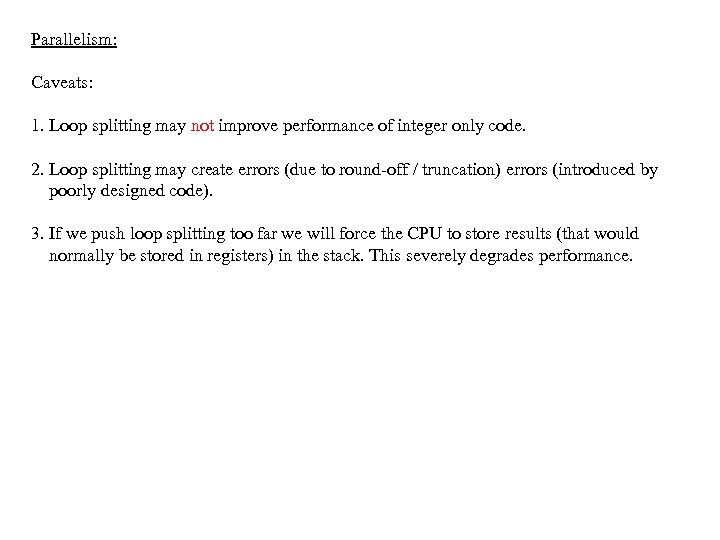Parallelism: Caveats: 1. Loop splitting may not improve performance of integer only code. 2. Loop splitting may create errors (due to round-off / truncation) errors (introduced by poorly designed code). 3. If we push loop splitting too far we will force the CPU to store results (that would normally be stored in registers) in the stack. This severely degrades performance.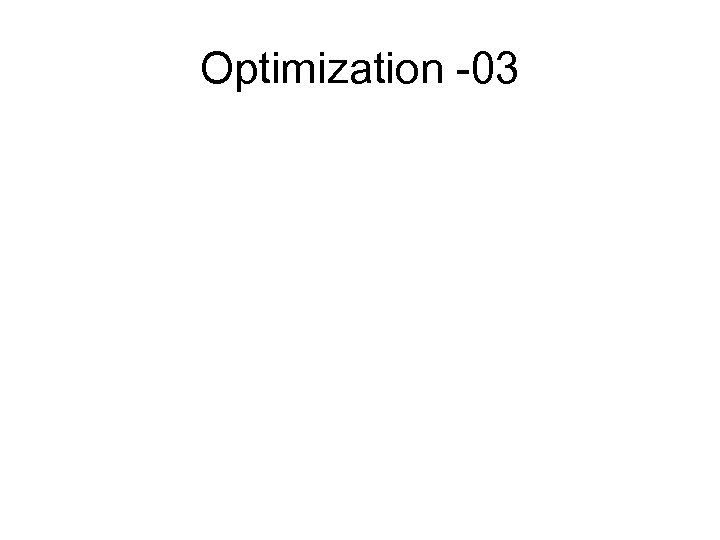Optimization -03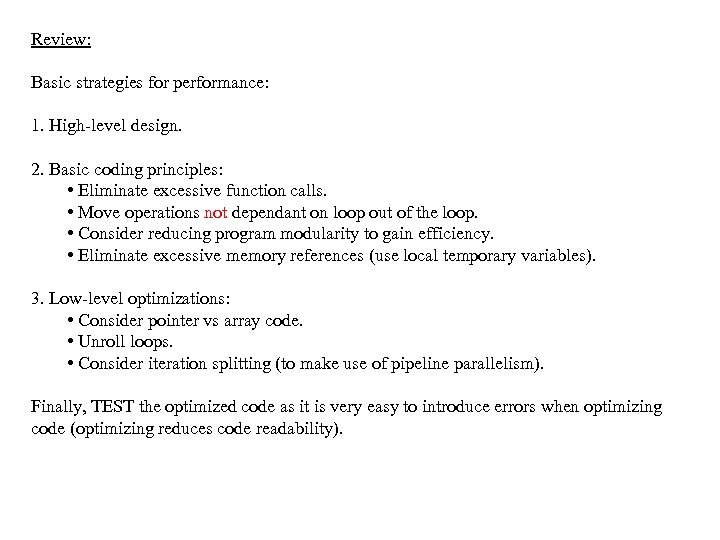Review: Basic strategies for performance: 1. High-level design. 2. Basic coding principles: • Eliminate excessive function calls. • Move operations not dependant on loop out of the loop. • Consider reducing program modularity to gain efficiency. • Eliminate excessive memory references (use local temporary variables). 3. Low-level optimizations: • Consider pointer vs array code. • Unroll loops. • Consider iteration splitting (to make use of pipeline parallelism). Finally, TEST the optimized code as it is very easy to introduce errors when optimizing code (optimizing reduces code readability).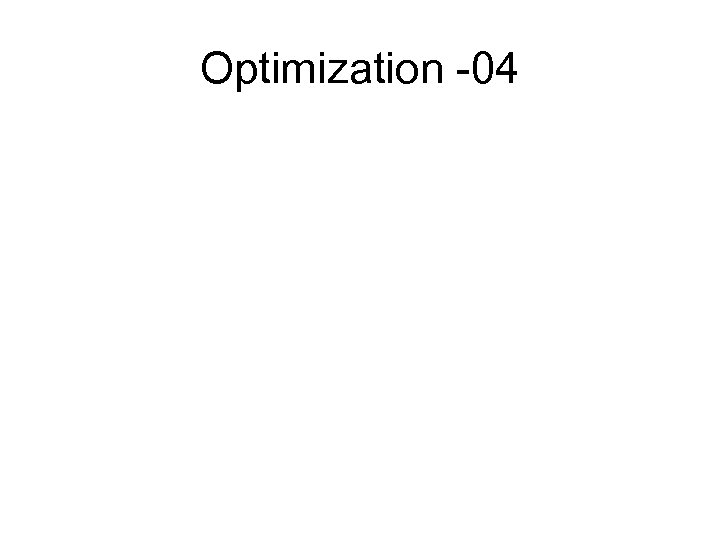Optimization -04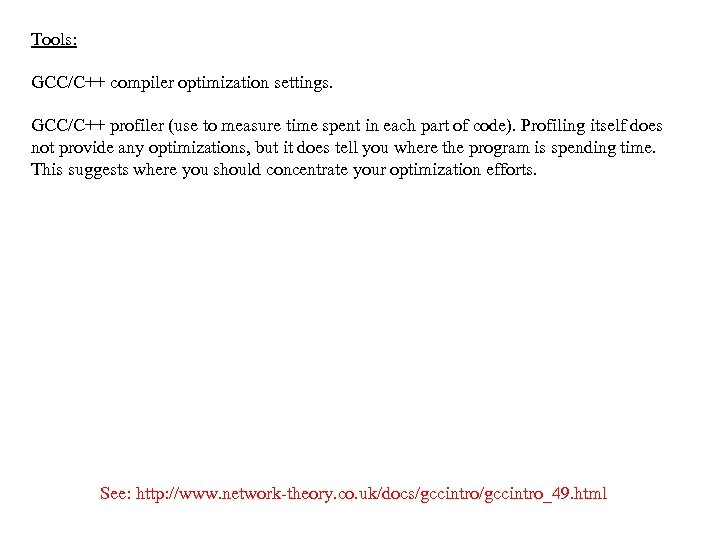Tools: GCC/C++ compiler optimization settings. GCC/C++ profiler (use to measure time spent in each part of code). Profiling itself does not provide any optimizations, but it does tell you where the program is spending time. This suggests where you should concentrate your optimization efforts. See: http: //www. network-theory. co. uk/docs/gccintro_49. html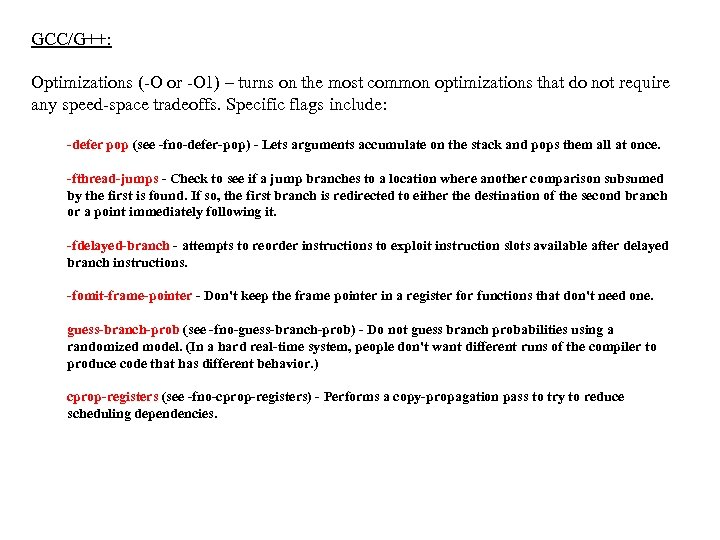GCC/G++: Optimizations (-O or -O 1) – turns on the most common optimizations that do not require any speed-space tradeoffs. Specific flags include: -defer pop (see -fno-defer-pop) - Lets arguments accumulate on the stack and pops them all at once. -fthread-jumps - Check to see if a jump branches to a location where another comparison subsumed by the first is found. If so, the first branch is redirected to either the destination of the second branch or a point immediately following it. -fdelayed-branch - attempts to reorder instructions to exploit instruction slots available after delayed branch instructions. -fomit-frame-pointer - Don't keep the frame pointer in a register for functions that don't need one. guess-branch-prob (see -fno-guess-branch-prob) - Do not guess branch probabilities using a randomized model. (In a hard real-time system, people don't want different runs of the compiler to produce code that has different behavior. ) cprop-registers (see -fno-cprop-registers) - Performs a copy-propagation pass to try to reduce scheduling dependencies.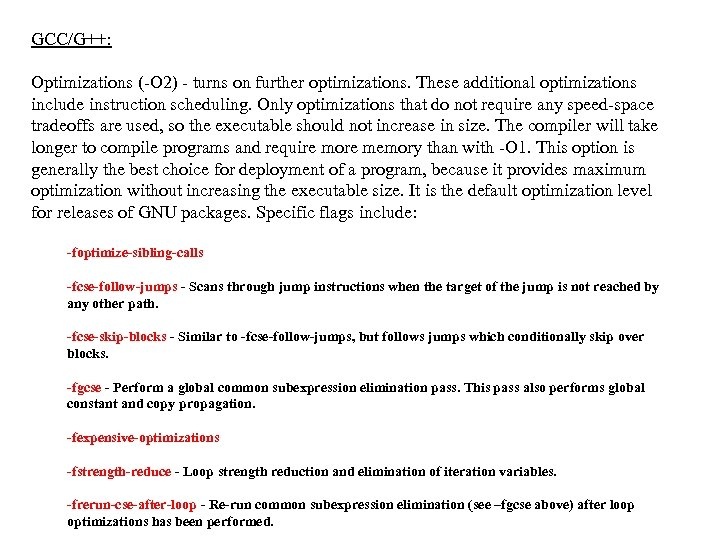GCC/G++: Optimizations (-O 2) - turns on further optimizations. These additional optimizations include instruction scheduling. Only optimizations that do not require any speed-space tradeoffs are used, so the executable should not increase in size. The compiler will take longer to compile programs and require more memory than with -O 1. This option is generally the best choice for deployment of a program, because it provides maximum optimization without increasing the executable size. It is the default optimization level for releases of GNU packages. Specific flags include: -foptimize-sibling-calls -fcse-follow-jumps - Scans through jump instructions when the target of the jump is not reached by any other path. -fcse-skip-blocks - Similar to -fcse-follow-jumps, but follows jumps which conditionally skip over blocks. -fgcse - Perform a global common subexpression elimination pass. This pass also performs global constant and copy propagation. -fexpensive-optimizations -fstrength-reduce - Loop strength reduction and elimination of iteration variables. -frerun-cse-after-loop - Re-run common subexpression elimination (see –fgcse above) after loop optimizations has been performed.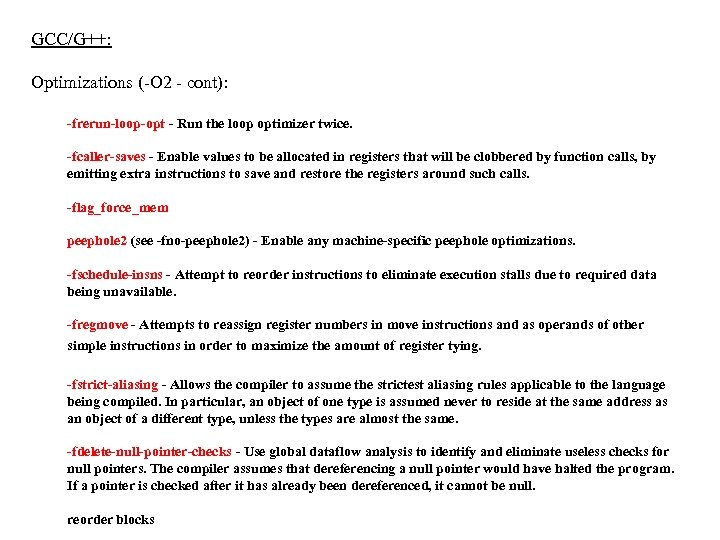GCC/G++: Optimizations (-O 2 - cont): -frerun-loop-opt - Run the loop optimizer twice. -fcaller-saves - Enable values to be allocated in registers that will be clobbered by function calls, by emitting extra instructions to save and restore the registers around such calls. -flag_force_mem peephole 2 (see -fno-peephole 2) - Enable any machine-specific peephole optimizations. -fschedule-insns - Attempt to reorder instructions to eliminate execution stalls due to required data being unavailable. -fregmove - Attempts to reassign register numbers in move instructions and as operands of other simple instructions in order to maximize the amount of register tying. -fstrict-aliasing - Allows the compiler to assume the strictest aliasing rules applicable to the language being compiled. In particular, an object of one type is assumed never to reside at the same address as an object of a different type, unless the types are almost the same. -fdelete-null-pointer-checks - Use global dataflow analysis to identify and eliminate useless checks for null pointers. The compiler assumes that dereferencing a null pointer would have halted the program. If a pointer is checked after it has already been dereferenced, it cannot be null. reorder blocks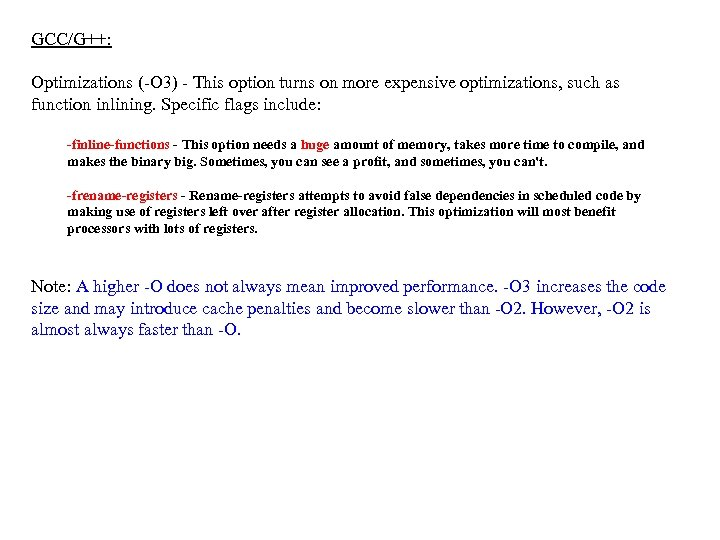GCC/G++: Optimizations (-O 3) - This option turns on more expensive optimizations, such as function inlining. Specific flags include: -finline-functions - This option needs a huge amount of memory, takes more time to compile, and makes the binary big. Sometimes, you can see a profit, and sometimes, you can't. -frename-registers - Rename-registers attempts to avoid false dependencies in scheduled code by making use of registers left over after register allocation. This optimization will most benefit processors with lots of registers. Note: A higher -O does not always mean improved performance. -O 3 increases the code size and may introduce cache penalties and become slower than -O 2. However, -O 2 is almost always faster than -O.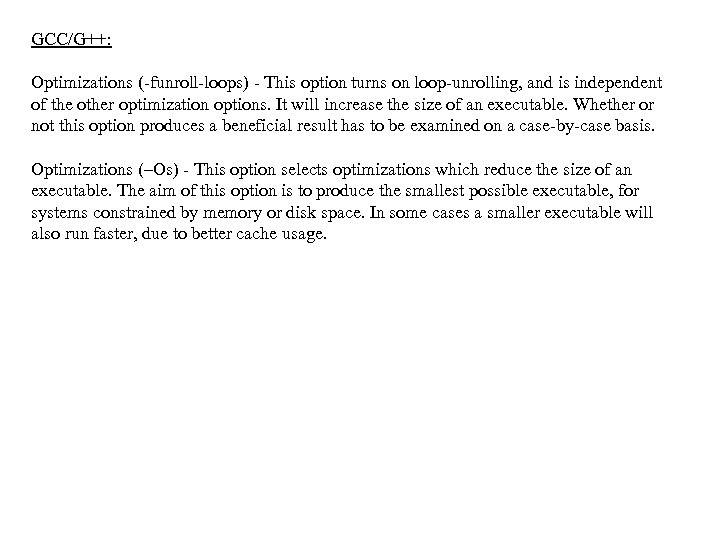GCC/G++: Optimizations (-funroll-loops) - This option turns on loop-unrolling, and is independent of the other optimization options. It will increase the size of an executable. Whether or not this option produces a beneficial result has to be examined on a case-by-case basis. Optimizations (–Os) - This option selects optimizations which reduce the size of an executable. The aim of this option is to produce the smallest possible executable, for systems constrained by memory or disk space. In some cases a smaller executable will also run faster, due to better cache usage.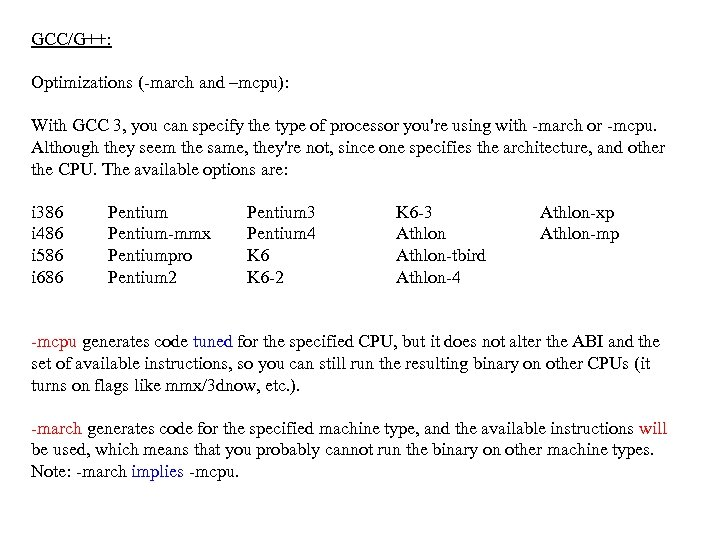GCC/G++: Optimizations (-march and –mcpu): With GCC 3, you can specify the type of processor you're using with -march or -mcpu. Although they seem the same, they're not, since one specifies the architecture, and other the CPU. The available options are: i 386 i 486 i 586 i 686 Pentium-mmx Pentiumpro Pentium 2 Pentium 3 Pentium 4 K 6 -2 K 6 -3 Athlon-tbird Athlon-4 Athlon-xp Athlon-mp -mcpu generates code tuned for the specified CPU, but it does not alter the ABI and the set of available instructions, so you can still run the resulting binary on other CPUs (it turns on flags like mmx/3 dnow, etc. ). -march generates code for the specified machine type, and the available instructions will be used, which means that you probably cannot run the binary on other machine types. Note: -march implies -mcpu.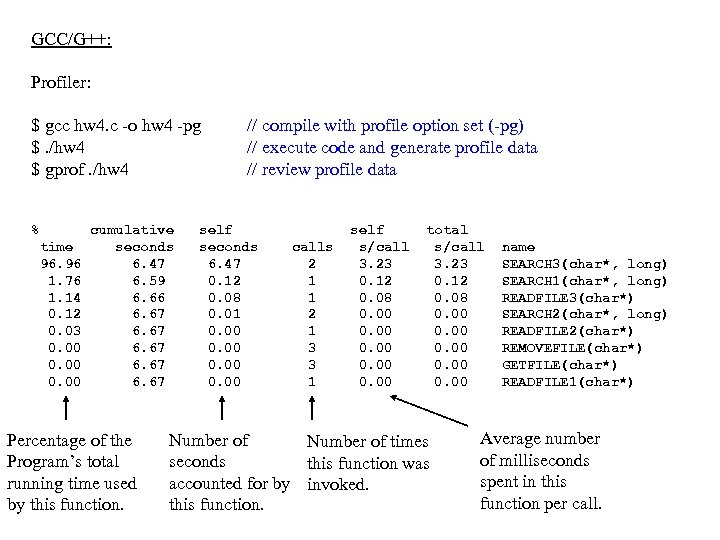GCC/G++: Profiler: \$ gcc hw 4. c -o hw 4 -pg \$. /hw 4 \$ gprof. /hw 4 % time 96. 96 1. 76 1. 14 0. 12 0. 03 0. 00 cumulative seconds 6. 47 6. 59 6. 66 6. 67 Percentage of the Program’s total running time used by this function. // compile with profile option set (-pg) // execute code and generate profile data // review profile data self seconds 6. 47 0. 12 0. 08 0. 01 0. 00 calls 2 1 1 2 1 3 3 1 self s/call 3. 23 0. 12 0. 08 0. 00 total s/call 3. 23 0. 12 0. 08 0. 00 Number of times seconds this function was accounted for by invoked. this function. name SEARCH 3(char*, long) SEARCH 1(char*, long) READFILE 3(char*) SEARCH 2(char*, long) READFILE 2(char*) REMOVEFILE(char*) GETFILE(char*) READFILE 1(char*) Average number of milliseconds spent in this function per call.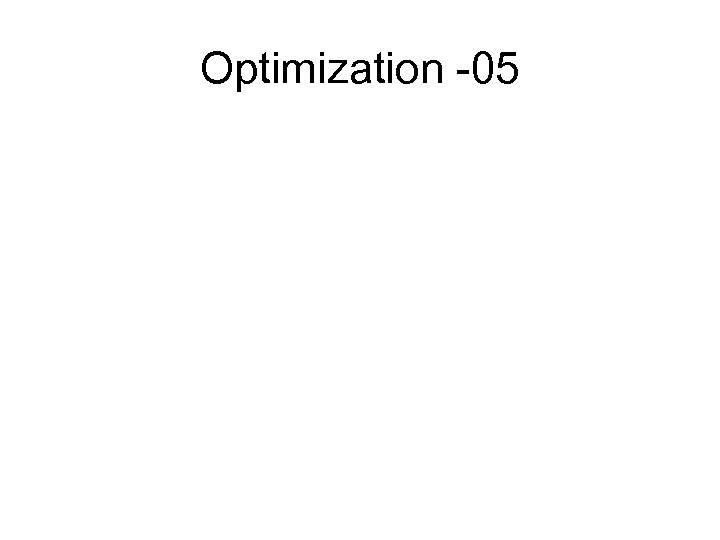Optimization -05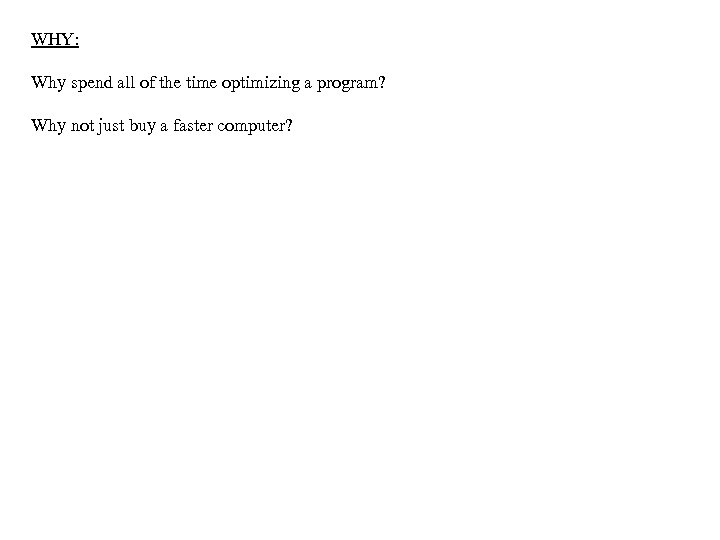WHY: Why spend all of the time optimizing a program? Why not just buy a faster computer?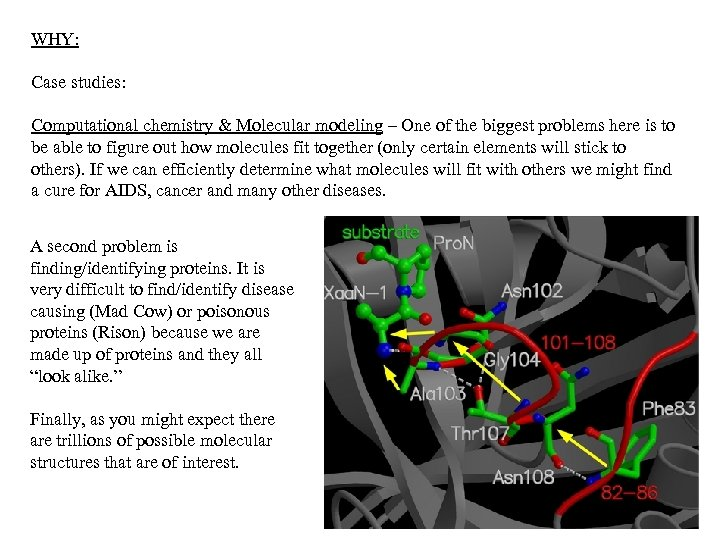WHY: Case studies: Computational chemistry & Molecular modeling – One of the biggest problems here is to be able to figure out how molecules fit together (only certain elements will stick to others). If we can efficiently determine what molecules will fit with others we might find a cure for AIDS, cancer and many other diseases. A second problem is finding/identifying proteins. It is very difficult to find/identify disease causing (Mad Cow) or poisonous proteins (Rison) because we are made up of proteins and they all “look alike. ” Finally, as you might expect there are trillions of possible molecular structures that are of interest.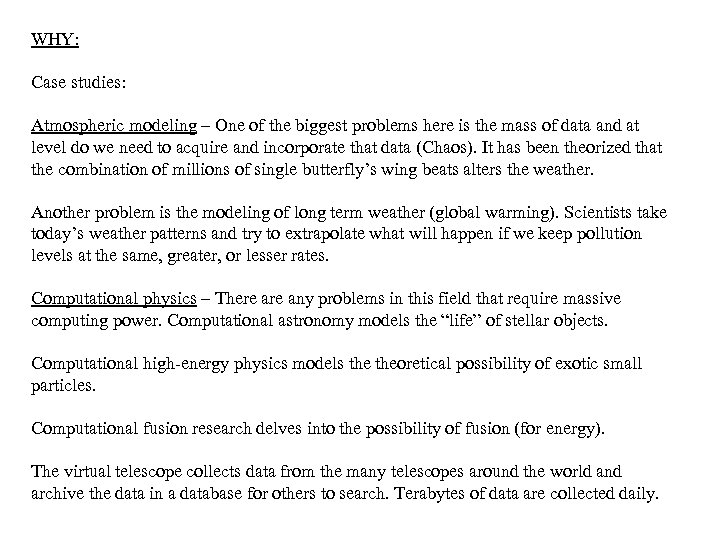WHY: Case studies: Atmospheric modeling – One of the biggest problems here is the mass of data and at level do we need to acquire and incorporate that data (Chaos). It has been theorized that the combination of millions of single butterfly’s wing beats alters the weather. Another problem is the modeling of long term weather (global warming). Scientists take today’s weather patterns and try to extrapolate what will happen if we keep pollution levels at the same, greater, or lesser rates. Computational physics – There any problems in this field that require massive computing power. Computational astronomy models the “life” of stellar objects. Computational high-energy physics models theoretical possibility of exotic small particles. Computational fusion research delves into the possibility of fusion (for energy). The virtual telescope collects data from the many telescopes around the world and archive the data in a database for others to search. Terabytes of data are collected daily.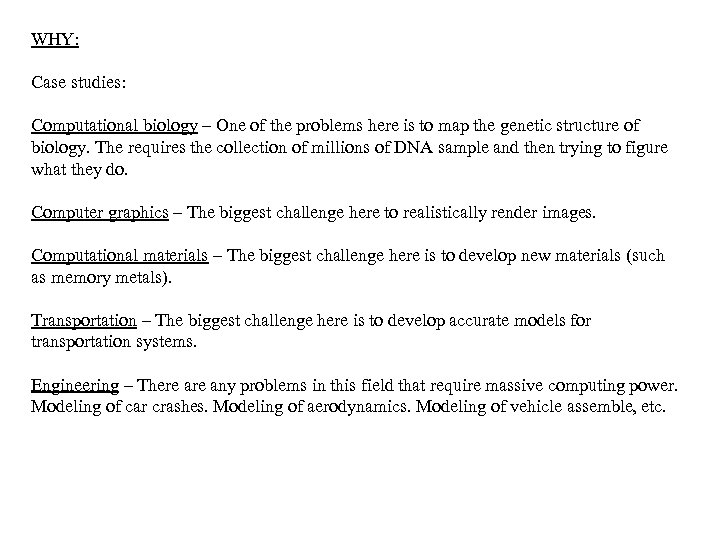WHY: Case studies: Computational biology – One of the problems here is to map the genetic structure of biology. The requires the collection of millions of DNA sample and then trying to figure what they do. Computer graphics – The biggest challenge here to realistically render images. Computational materials – The biggest challenge here is to develop new materials (such as memory metals). Transportation – The biggest challenge here is to develop accurate models for transportation systems. Engineering – There any problems in this field that require massive computing power. Modeling of car crashes. Modeling of aerodynamics. Modeling of vehicle assemble, etc.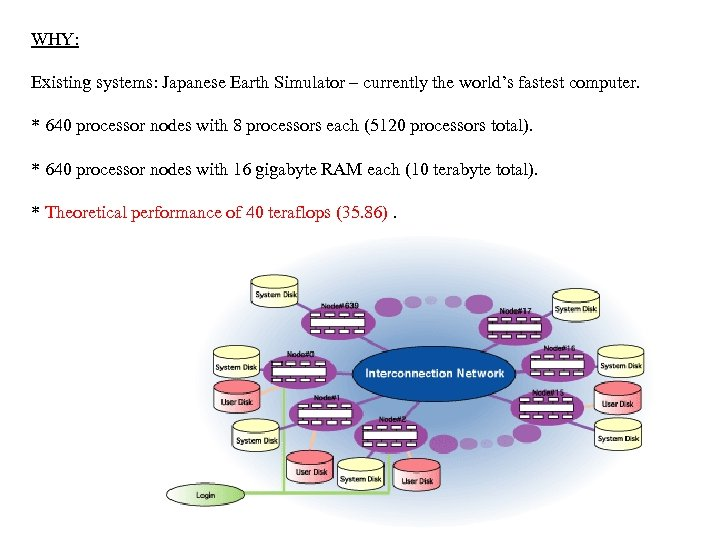WHY: Existing systems: Japanese Earth Simulator – currently the world’s fastest computer. * 640 processor nodes with 8 processors each (5120 processors total). * 640 processor nodes with 16 gigabyte RAM each (10 terabyte total). * Theoretical performance of 40 teraflops (35. 86).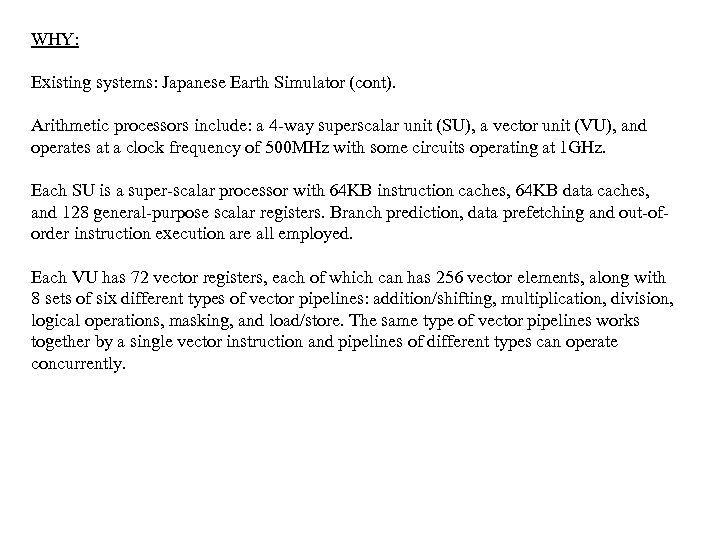WHY: Existing systems: Japanese Earth Simulator (cont). Arithmetic processors include: a 4 -way superscalar unit (SU), a vector unit (VU), and operates at a clock frequency of 500 MHz with some circuits operating at 1 GHz. Each SU is a super-scalar processor with 64 KB instruction caches, 64 KB data caches, and 128 general-purpose scalar registers. Branch prediction, data prefetching and out-oforder instruction execution are all employed. Each VU has 72 vector registers, each of which can has 256 vector elements, along with 8 sets of six different types of vector pipelines: addition/shifting, multiplication, division, logical operations, masking, and load/store. The same type of vector pipelines works together by a single vector instruction and pipelines of different types can operate concurrently.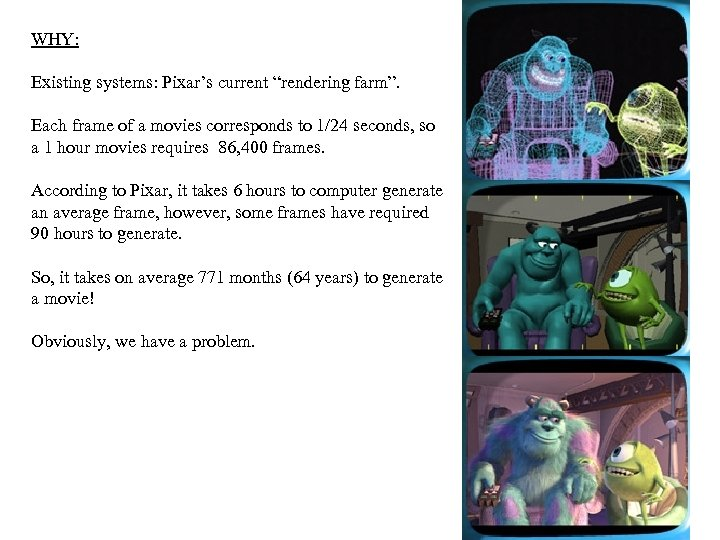WHY: Existing systems: Pixar’s current “rendering farm”. Each frame of a movies corresponds to 1/24 seconds, so a 1 hour movies requires 86, 400 frames. According to Pixar, it takes 6 hours to computer generate an average frame, however, some frames have required 90 hours to generate. So, it takes on average 771 months (64 years) to generate a movie! Obviously, we have a problem.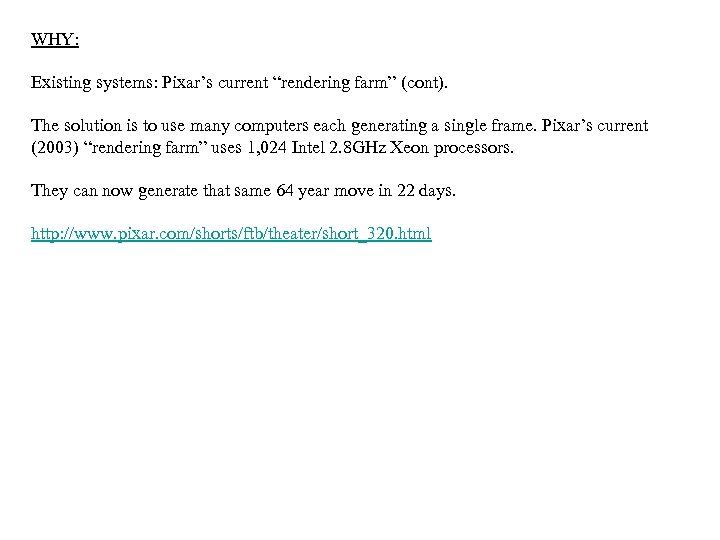WHY: Existing systems: Pixar’s current “rendering farm” (cont). The solution is to use many computers each generating a single frame. Pixar’s current (2003) “rendering farm” uses 1, 024 Intel 2. 8 GHz Xeon processors. They can now generate that same 64 year move in 22 days. http: //www. pixar. com/shorts/ftb/theater/short_320. html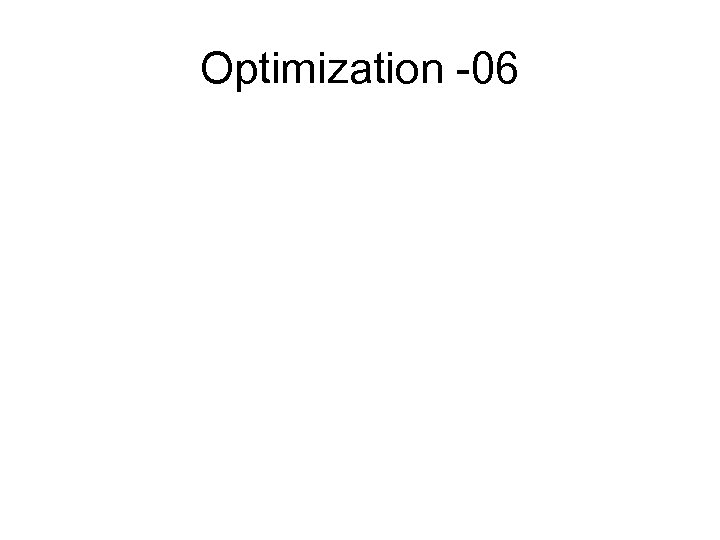Optimization -06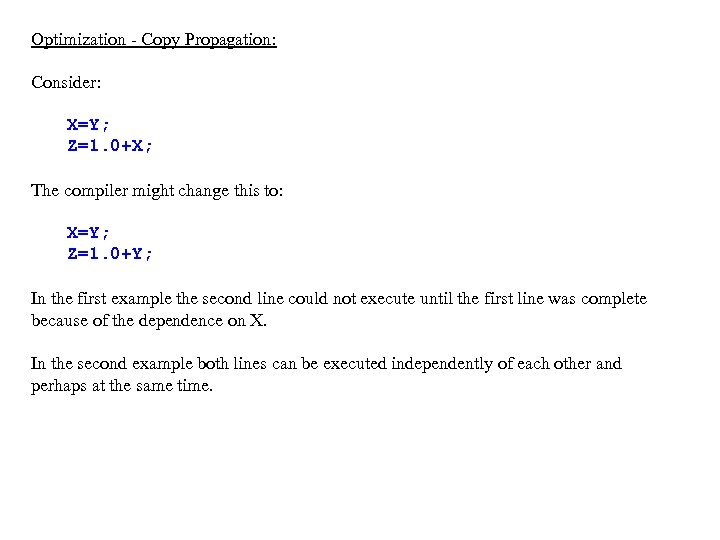Optimization - Copy Propagation: Consider: X=Y; Z=1. 0+X; The compiler might change this to: X=Y; Z=1. 0+Y; In the first example the second line could not execute until the first line was complete because of the dependence on X. In the second example both lines can be executed independently of each other and perhaps at the same time.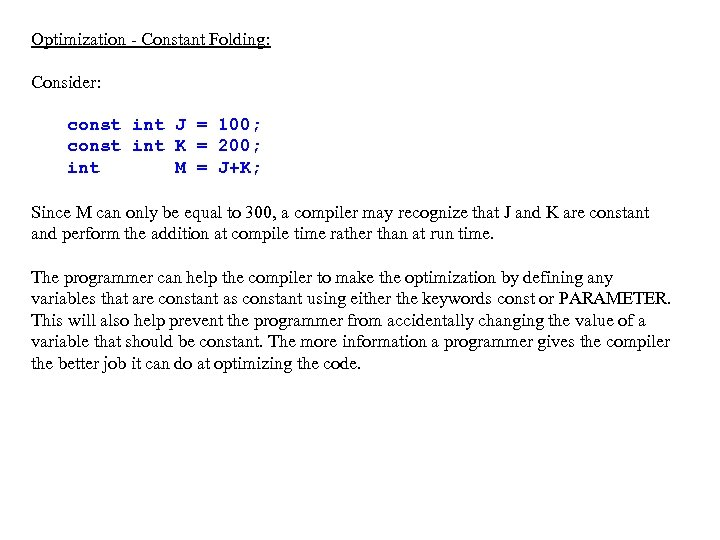Optimization - Constant Folding: Consider: const int J = 100; const int K = 200; int M = J+K; Since M can only be equal to 300, a compiler may recognize that J and K are constant and perform the addition at compile time rather than at run time. The programmer can help the compiler to make the optimization by defining any variables that are constant as constant using either the keywords const or PARAMETER. This will also help prevent the programmer from accidentally changing the value of a variable that should be constant. The more information a programmer gives the compiler the better job it can do at optimizing the code.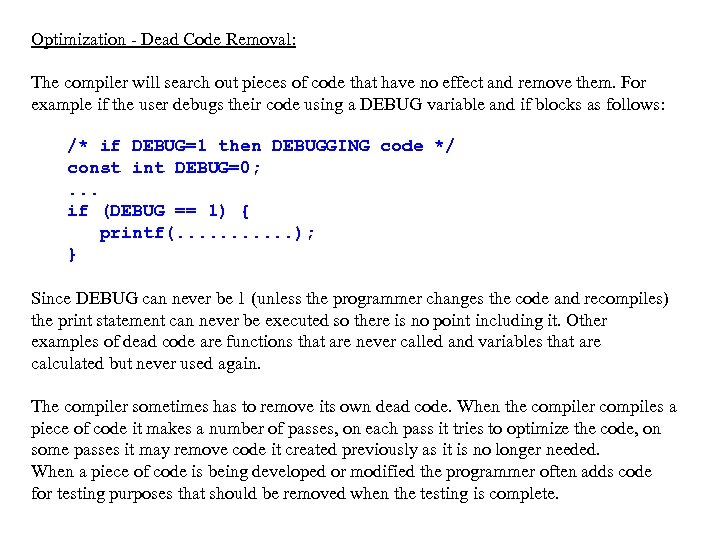Optimization - Dead Code Removal: The compiler will search out pieces of code that have no effect and remove them. For example if the user debugs their code using a DEBUG variable and if blocks as follows: /* if DEBUG=1 then DEBUGGING code */ const int DEBUG=0; . . . if (DEBUG == 1) { printf(. . . ); } Since DEBUG can never be 1 (unless the programmer changes the code and recompiles) the print statement can never be executed so there is no point including it. Other examples of dead code are functions that are never called and variables that are calculated but never used again. The compiler sometimes has to remove its own dead code. When the compiler compiles a piece of code it makes a number of passes, on each pass it tries to optimize the code, on some passes it may remove code it created previously as it is no longer needed. When a piece of code is being developed or modified the programmer often adds code for testing purposes that should be removed when the testing is complete.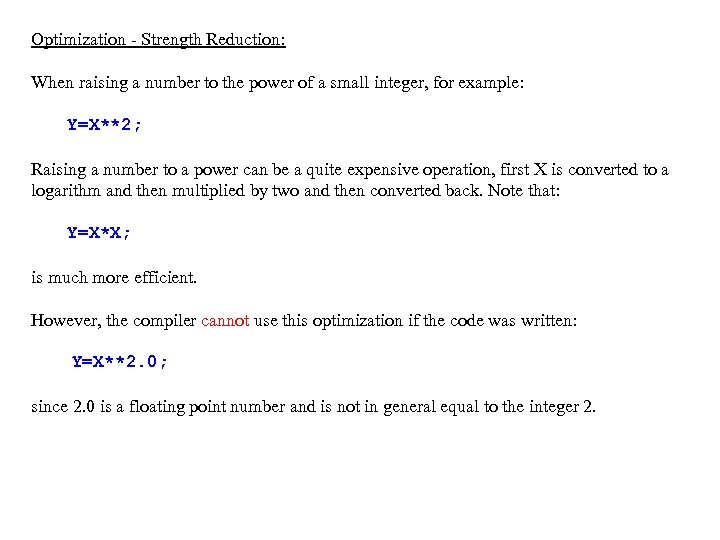Optimization - Strength Reduction: When raising a number to the power of a small integer, for example: Y=X**2; Raising a number to a power can be a quite expensive operation, first X is converted to a logarithm and then multiplied by two and then converted back. Note that: Y=X*X; is much more efficient. However, the compiler cannot use this optimization if the code was written: Y=X**2. 0; since 2. 0 is a floating point number and is not in general equal to the integer 2.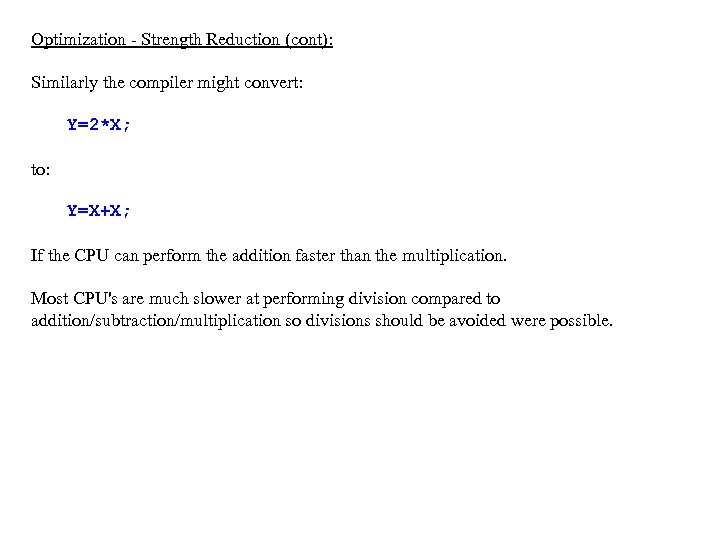Optimization - Strength Reduction (cont): Similarly the compiler might convert: Y=2*X; to: Y=X+X; If the CPU can perform the addition faster than the multiplication. Most CPU's are much slower at performing division compared to addition/subtraction/multiplication so divisions should be avoided were possible.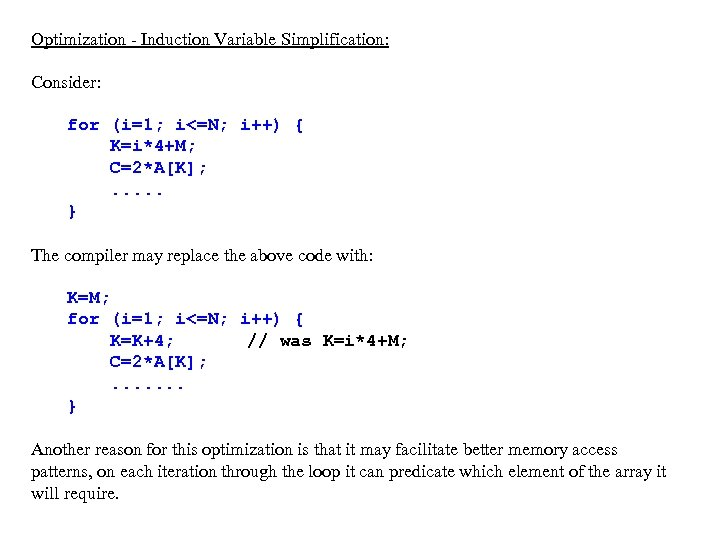Optimization - Induction Variable Simplification: Consider: for (i=1; i<=N; i++) { K=i*4+M; C=2*A[K]; . . . } The compiler may replace the above code with: K=M; for (i=1; i<=N; i++) { K=K+4; // was K=i*4+M; C=2*A[K]; . . . . } Another reason for this optimization is that it may facilitate better memory access patterns, on each iteration through the loop it can predicate which element of the array it will require.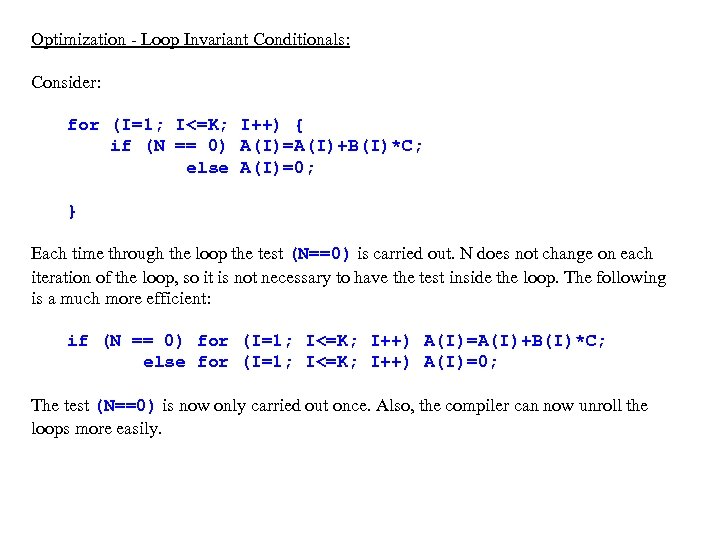Optimization - Loop Invariant Conditionals: Consider: for (I=1; I<=K; I++) { if (N == 0) A(I)=A(I)+B(I)*C; else A(I)=0; } Each time through the loop the test (N==0) is carried out. N does not change on each iteration of the loop, so it is not necessary to have the test inside the loop. The following is a much more efficient: if (N == 0) for (I=1; I<=K; I++) A(I)=A(I)+B(I)*C; else for (I=1; I<=K; I++) A(I)=0; The test (N==0) is now only carried out once. Also, the compiler can now unroll the loops more easily.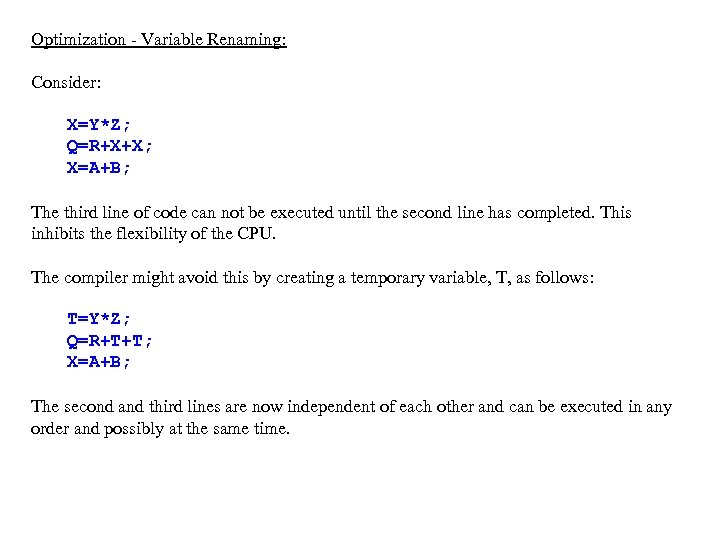Optimization - Variable Renaming: Consider: X=Y*Z; Q=R+X+X; X=A+B; The third line of code can not be executed until the second line has completed. This inhibits the flexibility of the CPU. The compiler might avoid this by creating a temporary variable, T, as follows: T=Y*Z; Q=R+T+T; X=A+B; The second and third lines are now independent of each other and can be executed in any order and possibly at the same time.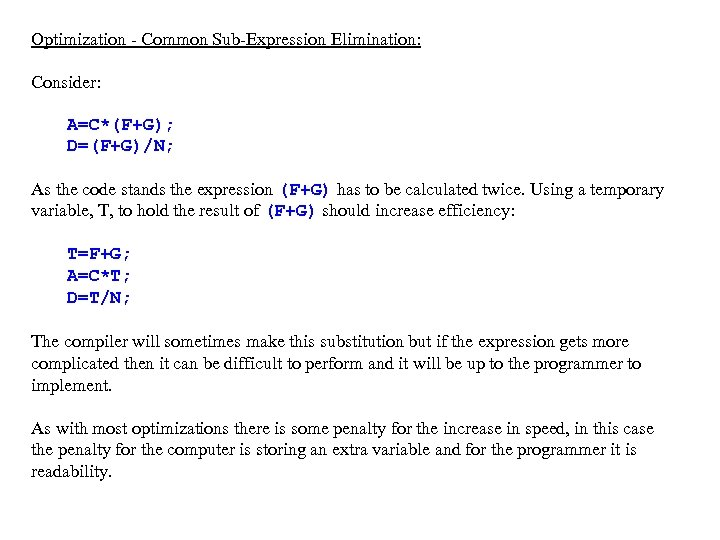Optimization - Common Sub-Expression Elimination: Consider: A=C*(F+G); D=(F+G)/N; As the code stands the expression (F+G) has to be calculated twice. Using a temporary variable, T, to hold the result of (F+G) should increase efficiency: T=F+G; A=C*T; D=T/N; The compiler will sometimes make this substitution but if the expression gets more complicated then it can be difficult to perform and it will be up to the programmer to implement. As with most optimizations there is some penalty for the increase in speed, in this case the penalty for the computer is storing an extra variable and for the programmer it is readability.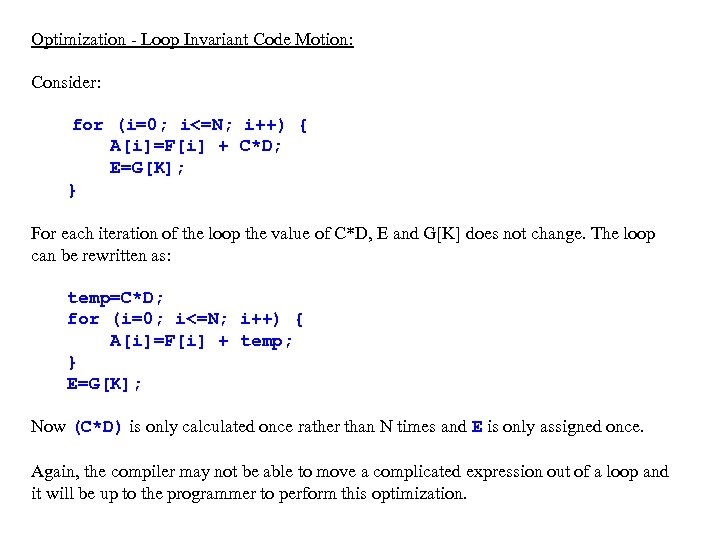Optimization - Loop Invariant Code Motion: Consider: for (i=0; i<=N; i++) { A[i]=F[i] + C*D; E=G[K]; } For each iteration of the loop the value of C*D, E and G[K] does not change. The loop can be rewritten as: temp=C*D; for (i=0; i<=N; i++) { A[i]=F[i] + temp; } E=G[K]; Now (C*D) is only calculated once rather than N times and E is only assigned once. Again, the compiler may not be able to move a complicated expression out of a loop and it will be up to the programmer to perform this optimization.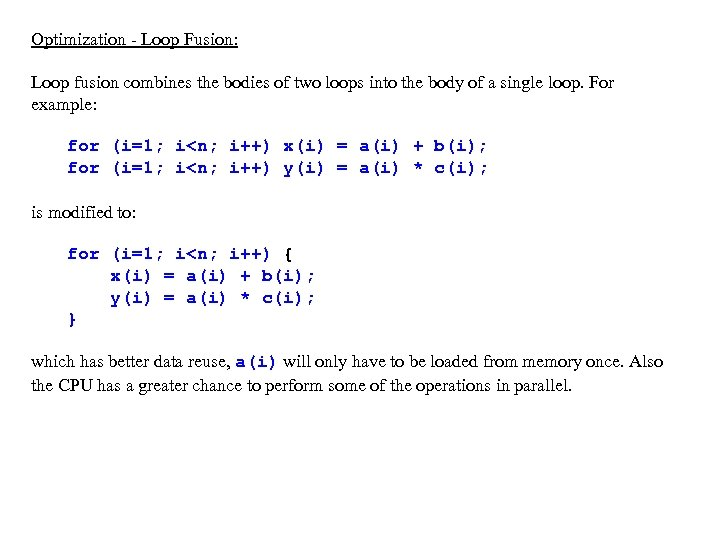Optimization - Loop Fusion: Loop fusion combines the bodies of two loops into the body of a single loop. For example: for (i=1; i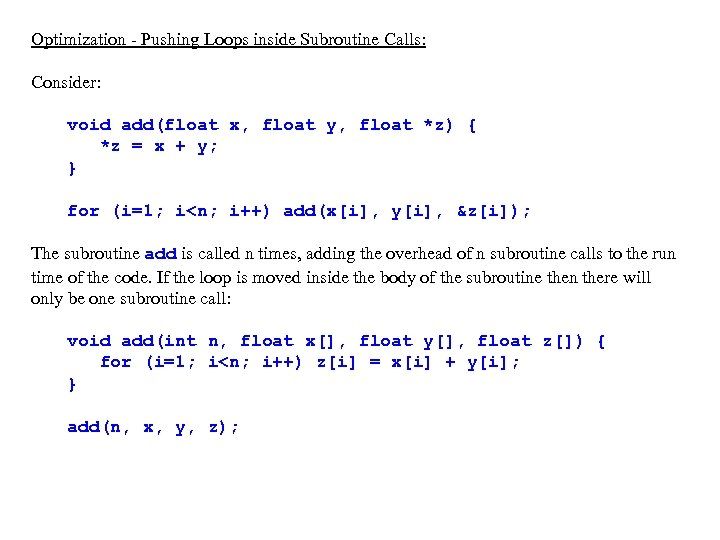Optimization - Pushing Loops inside Subroutine Calls: Consider: void add(float x, float y, float *z) { *z = x + y; } for (i=1; i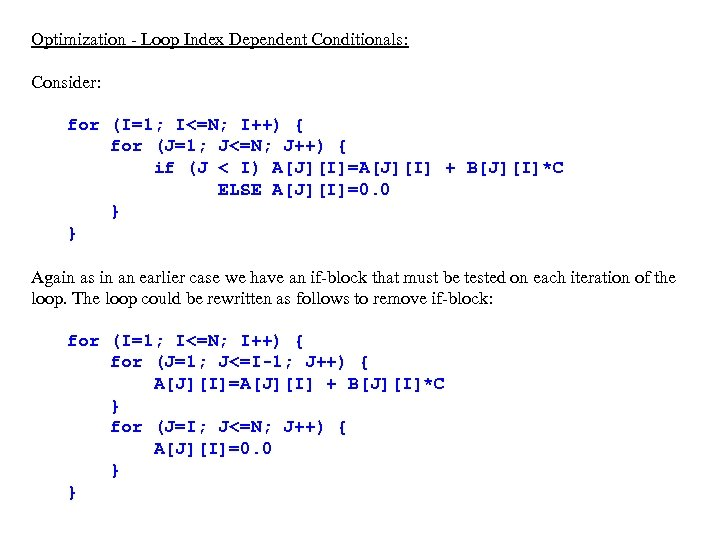Optimization - Loop Index Dependent Conditionals: Consider: for (I=1; I<=N; I++) { for (J=1; J<=N; J++) { if (J < I) A[J][I]=A[J][I] + B[J][I]*C ELSE A[J][I]=0. 0 } } Again as in an earlier case we have an if-block that must be tested on each iteration of the loop. The loop could be rewritten as follows to remove if-block: for (I=1; I<=N; I++) { for (J=1; J<=I-1; J++) { A[J][I]=A[J][I] + B[J][I]*C } for (J=I; J<=N; J++) { A[J][I]=0. 0 } }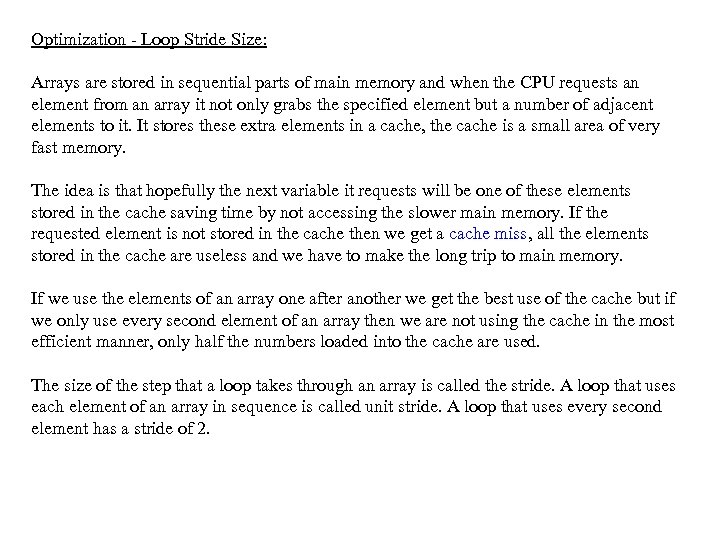Optimization - Loop Stride Size: Arrays are stored in sequential parts of main memory and when the CPU requests an element from an array it not only grabs the specified element but a number of adjacent elements to it. It stores these extra elements in a cache, the cache is a small area of very fast memory. The idea is that hopefully the next variable it requests will be one of these elements stored in the cache saving time by not accessing the slower main memory. If the requested element is not stored in the cache then we get a cache miss, all the elements stored in the cache are useless and we have to make the long trip to main memory. If we use the elements of an array one after another we get the best use of the cache but if we only use every second element of an array then we are not using the cache in the most efficient manner, only half the numbers loaded into the cache are used. The size of the step that a loop takes through an array is called the stride. A loop that uses each element of an array in sequence is called unit stride. A loop that uses every second element has a stride of 2.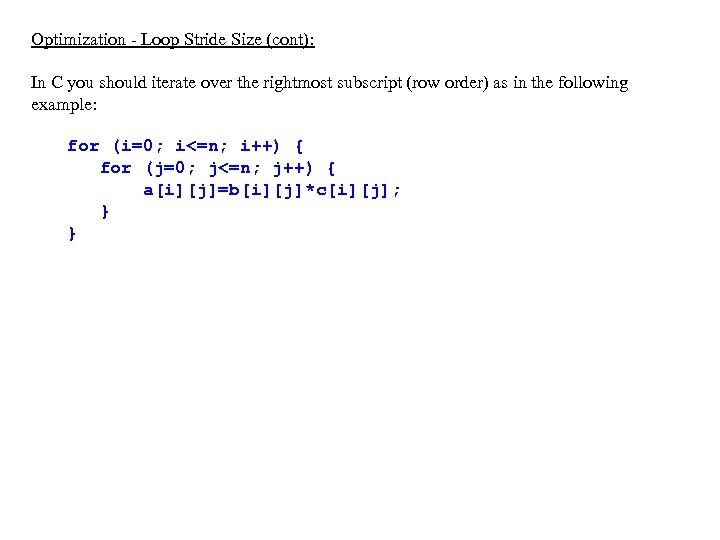Optimization - Loop Stride Size (cont): In C you should iterate over the rightmost subscript (row order) as in the following example: for (i=0; i<=n; i++) { for (j=0; j<=n; j++) { a[i][j]=b[i][j]*c[i][j]; } }